# RS Aggarwal Solutions for Class 9 Chapter 11: Areas of Parallelograms and Triangles

The solutions for Class 9 Maths are prepared by highly knowledgeable expert faculties based on the current CBSE syllabus. RS Aggarwal Solutions for Class 9 contain answers in PDF format which can be downloaded by the students and used as a reference material. The students need to understand the concepts of Class 9 thoroughly as some of them will be continued in Class 10 as well.

The students can use the RS Aggarwal solutions PDF to understand other ways to solve the same problem faster. The solutions contain various methods of solving the same problem to help students with time management. RS Aggarwal Solutions for Class 9 Chapter 11 Areas of Parallelograms and Triangles are provided here.

## RS Aggarwal Solutions for Class 9 Chapter 11: Areas of Parallelograms and Triangles Download PDF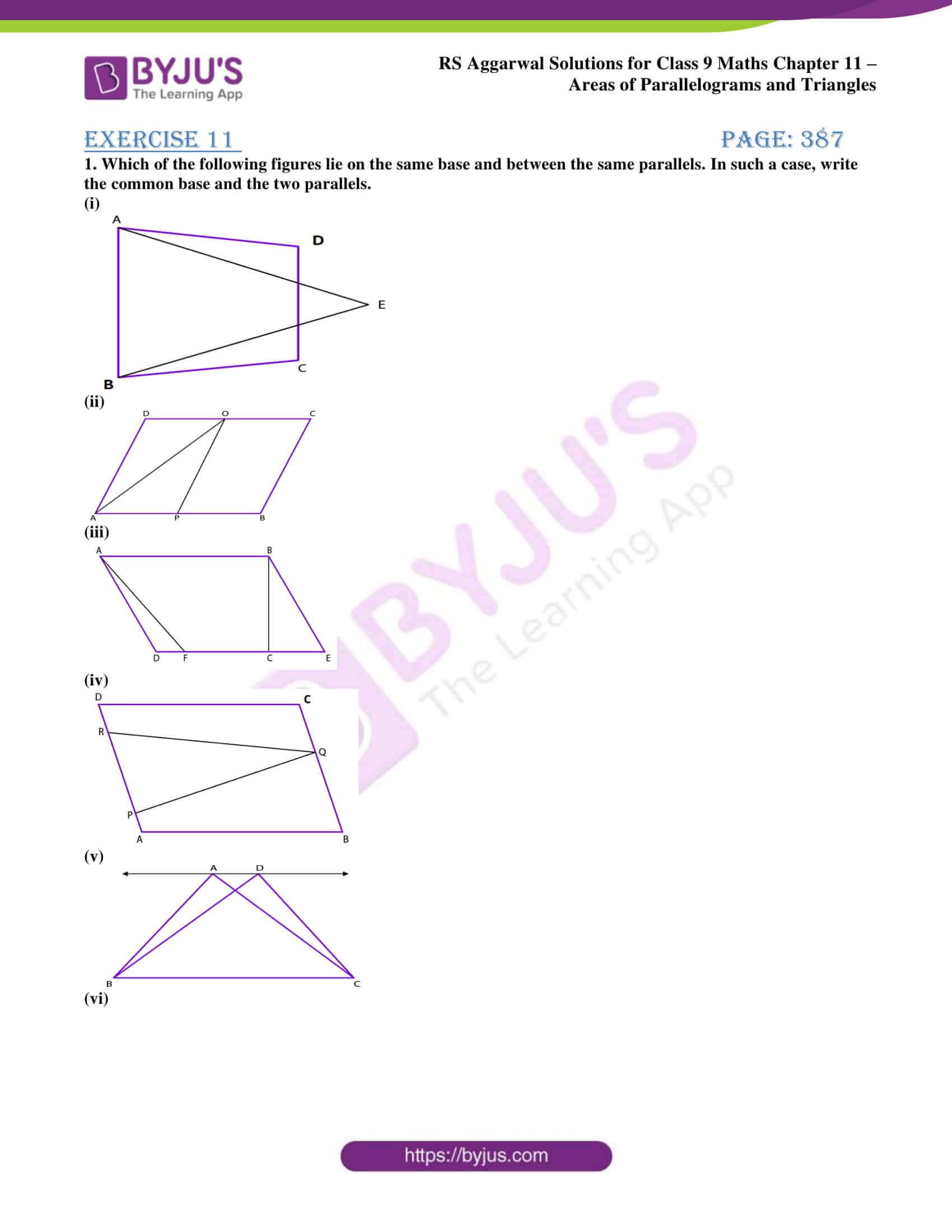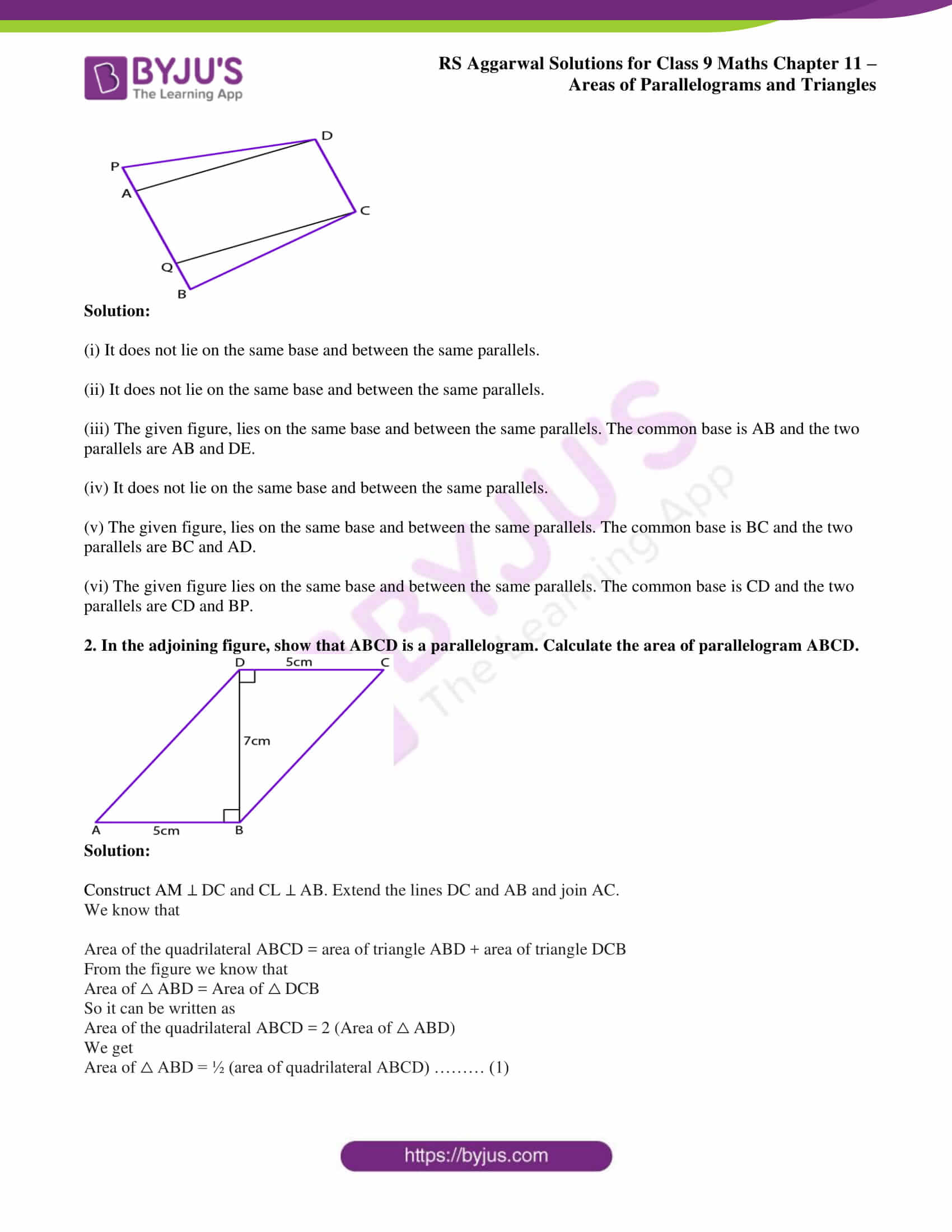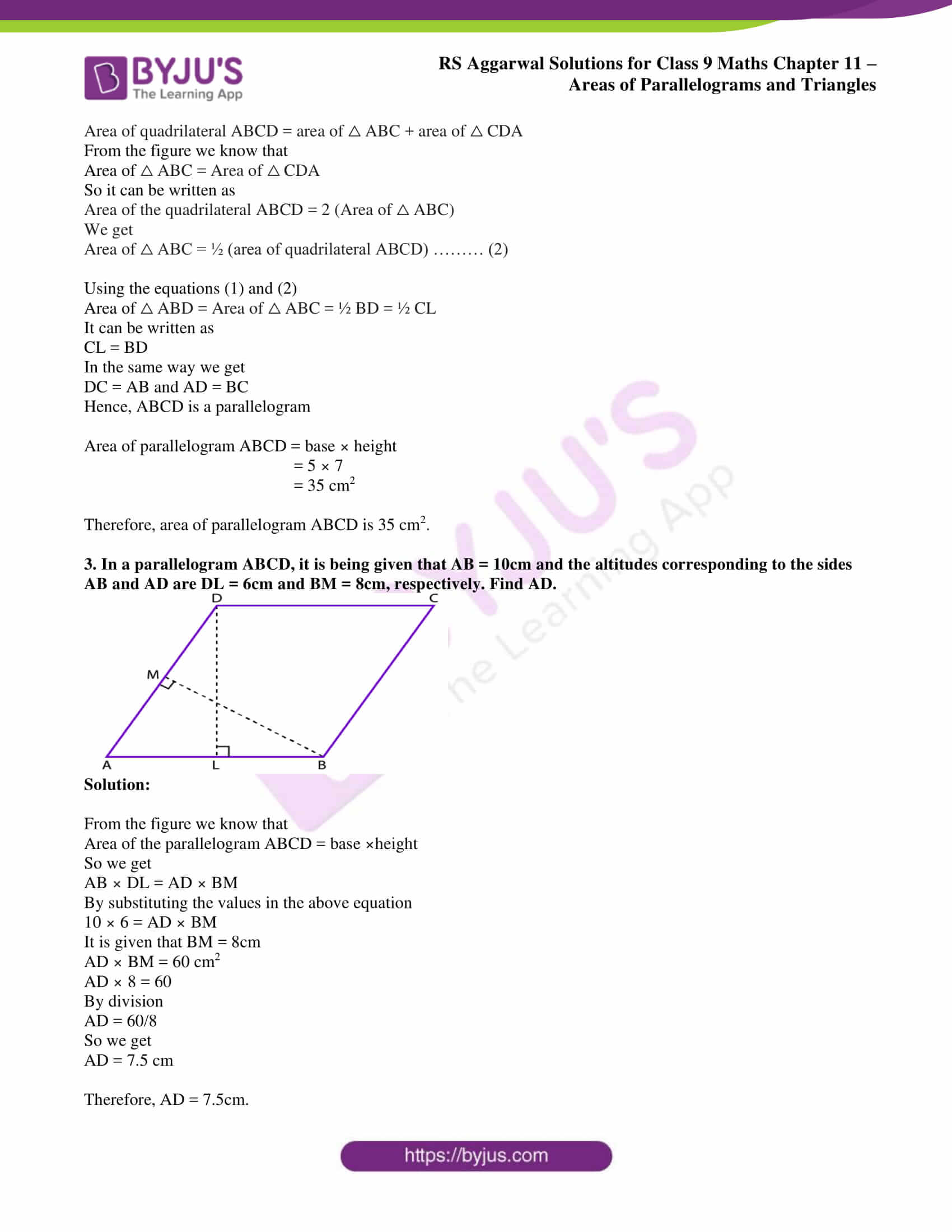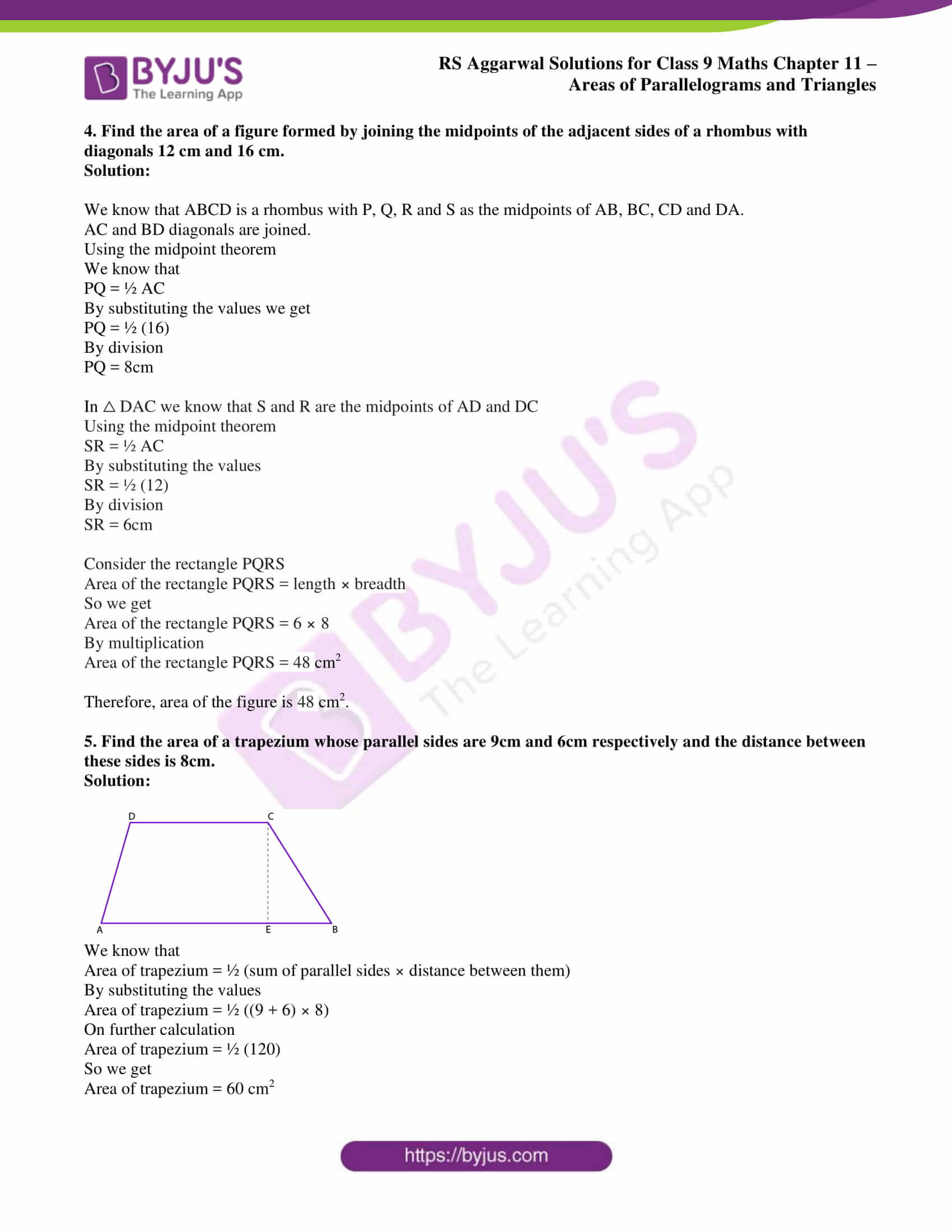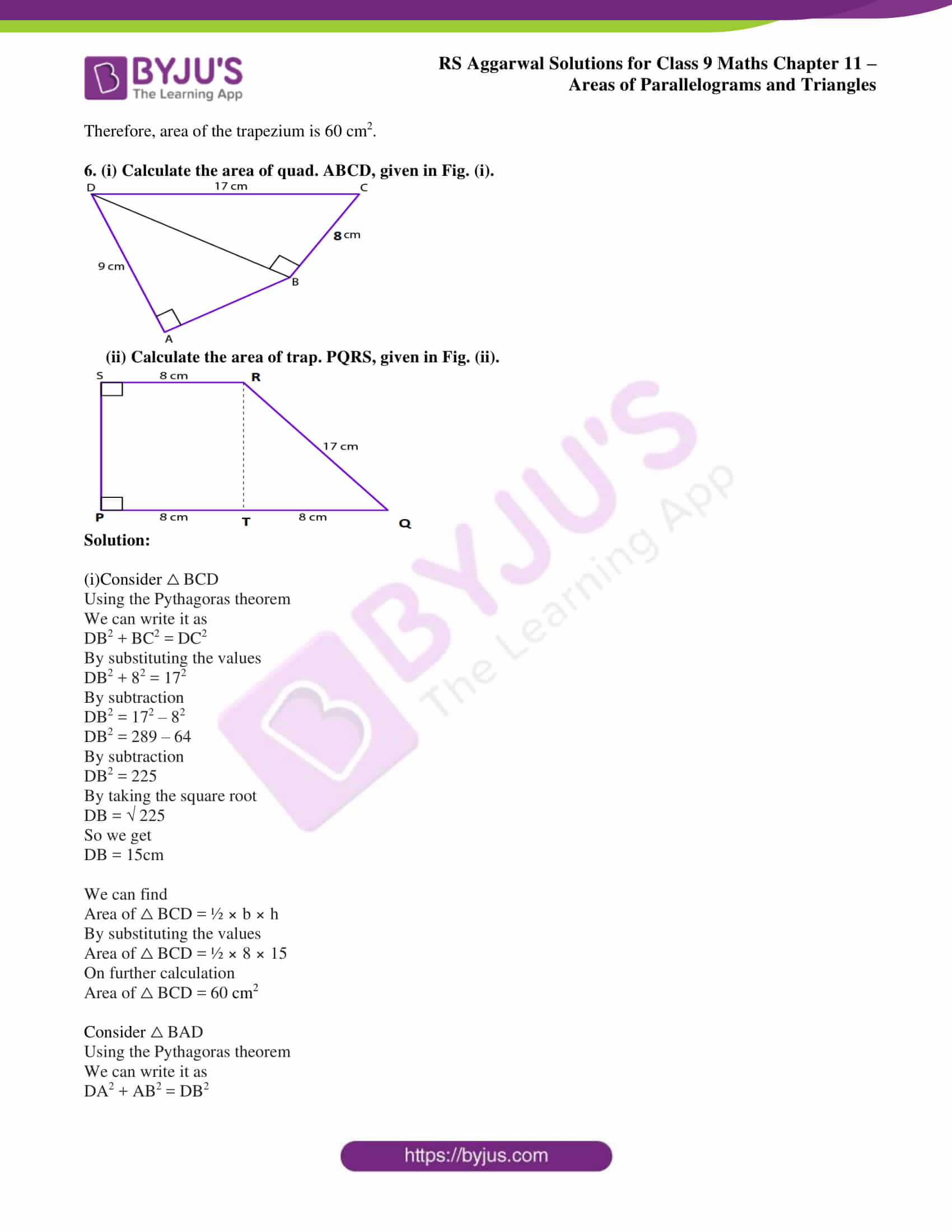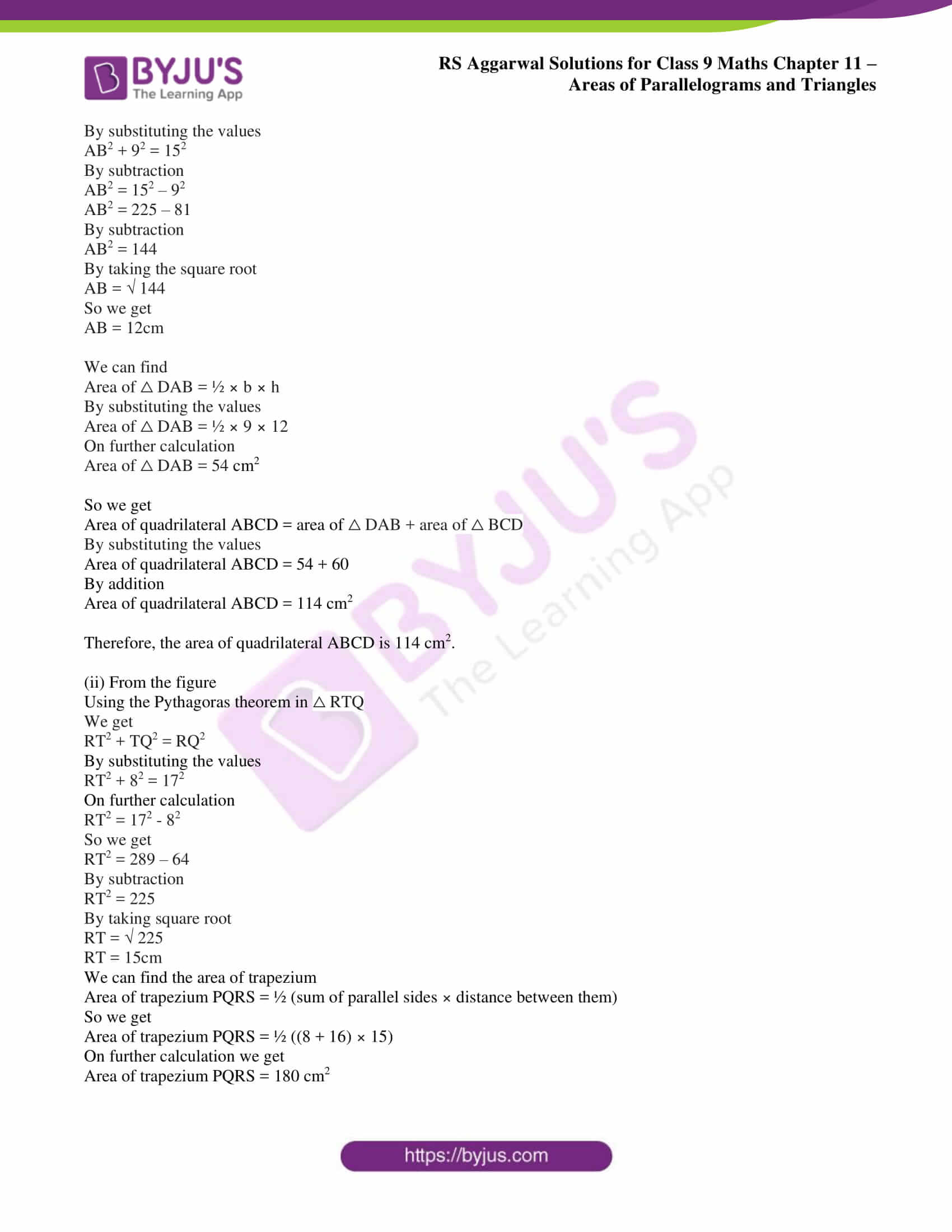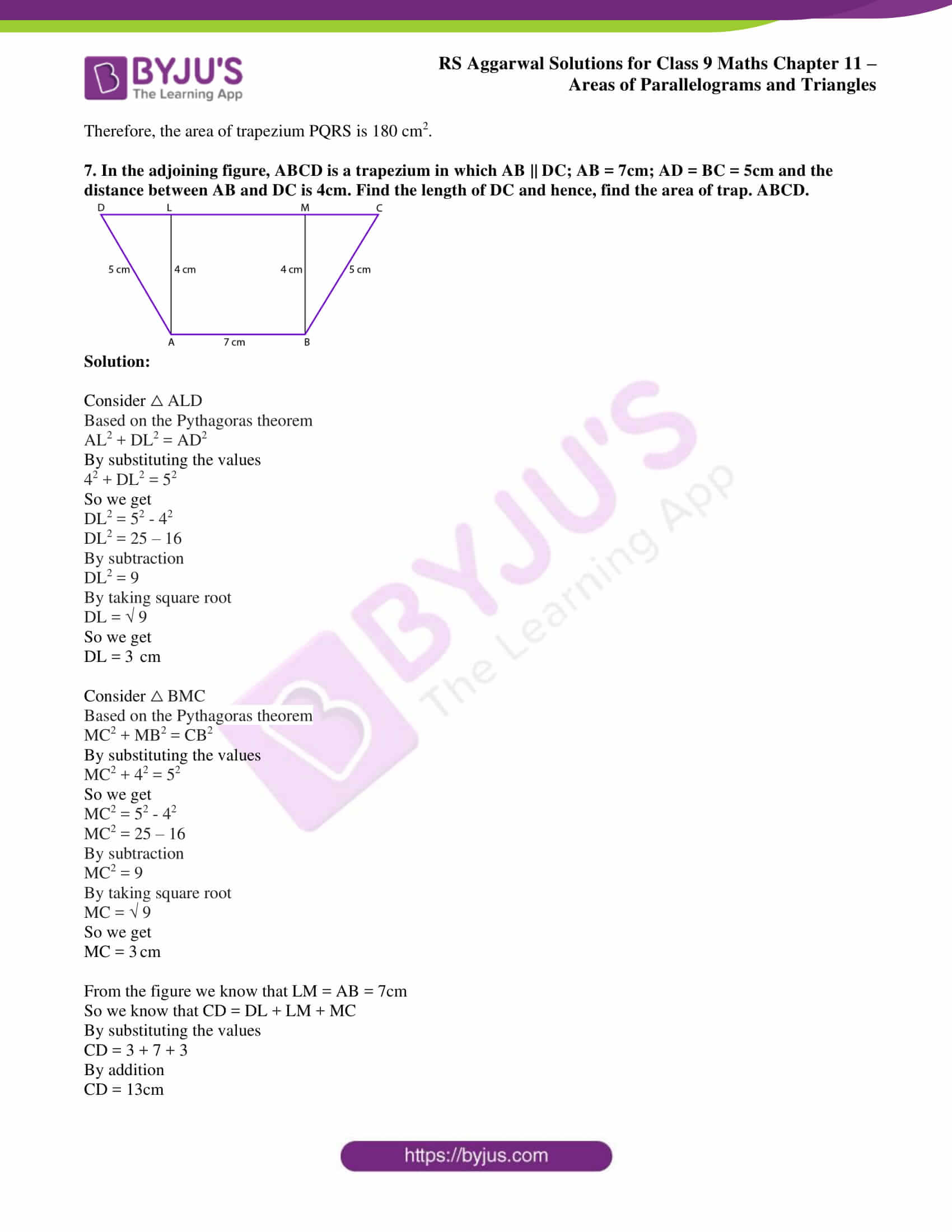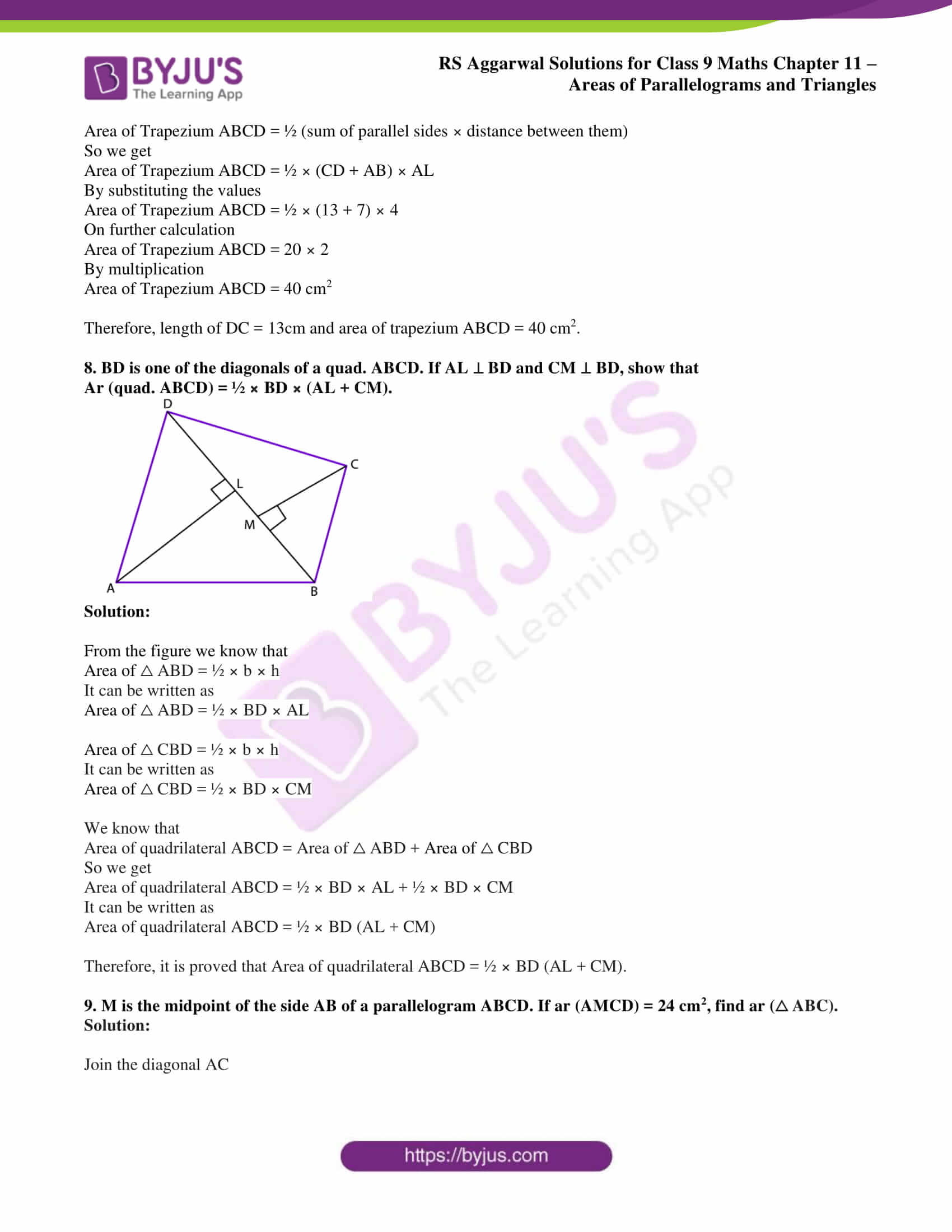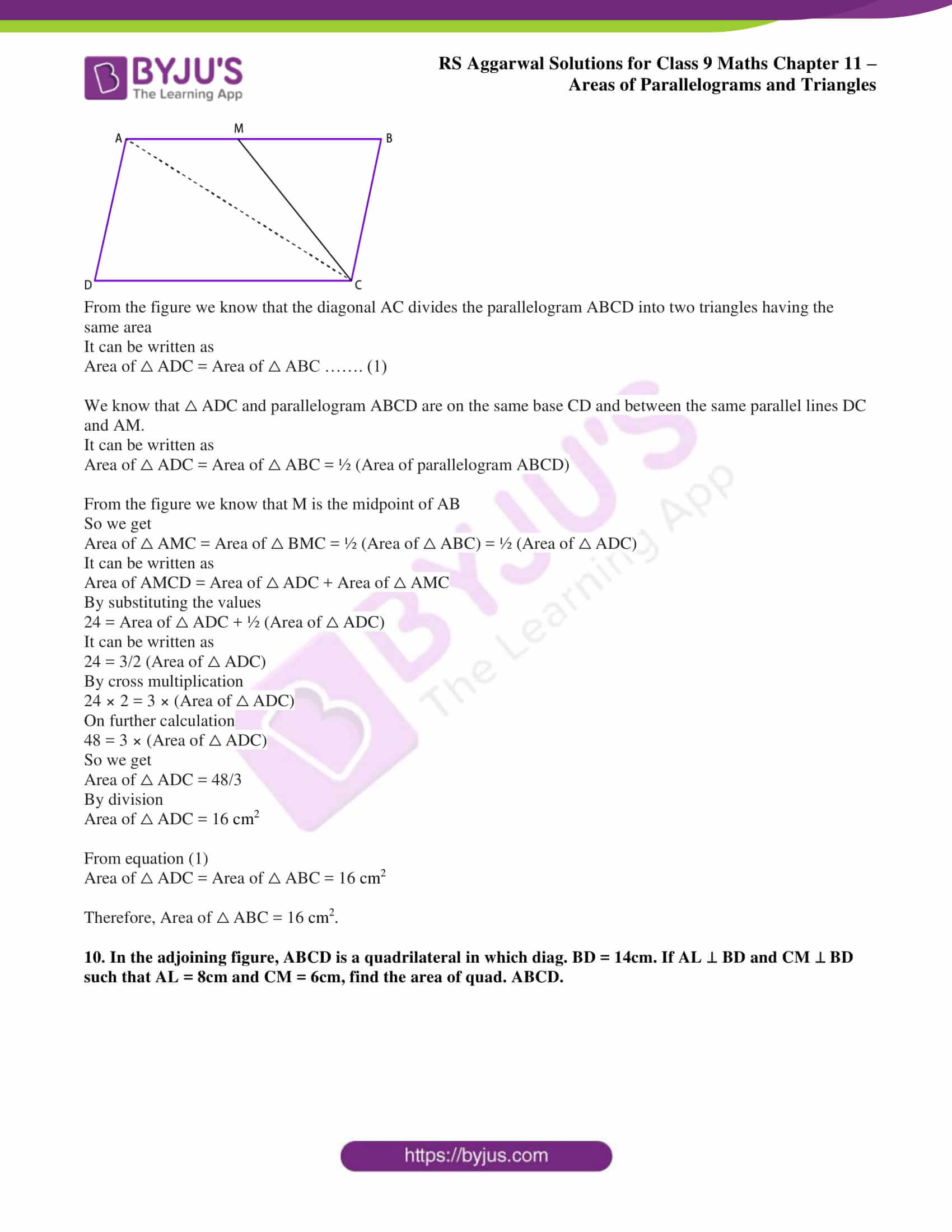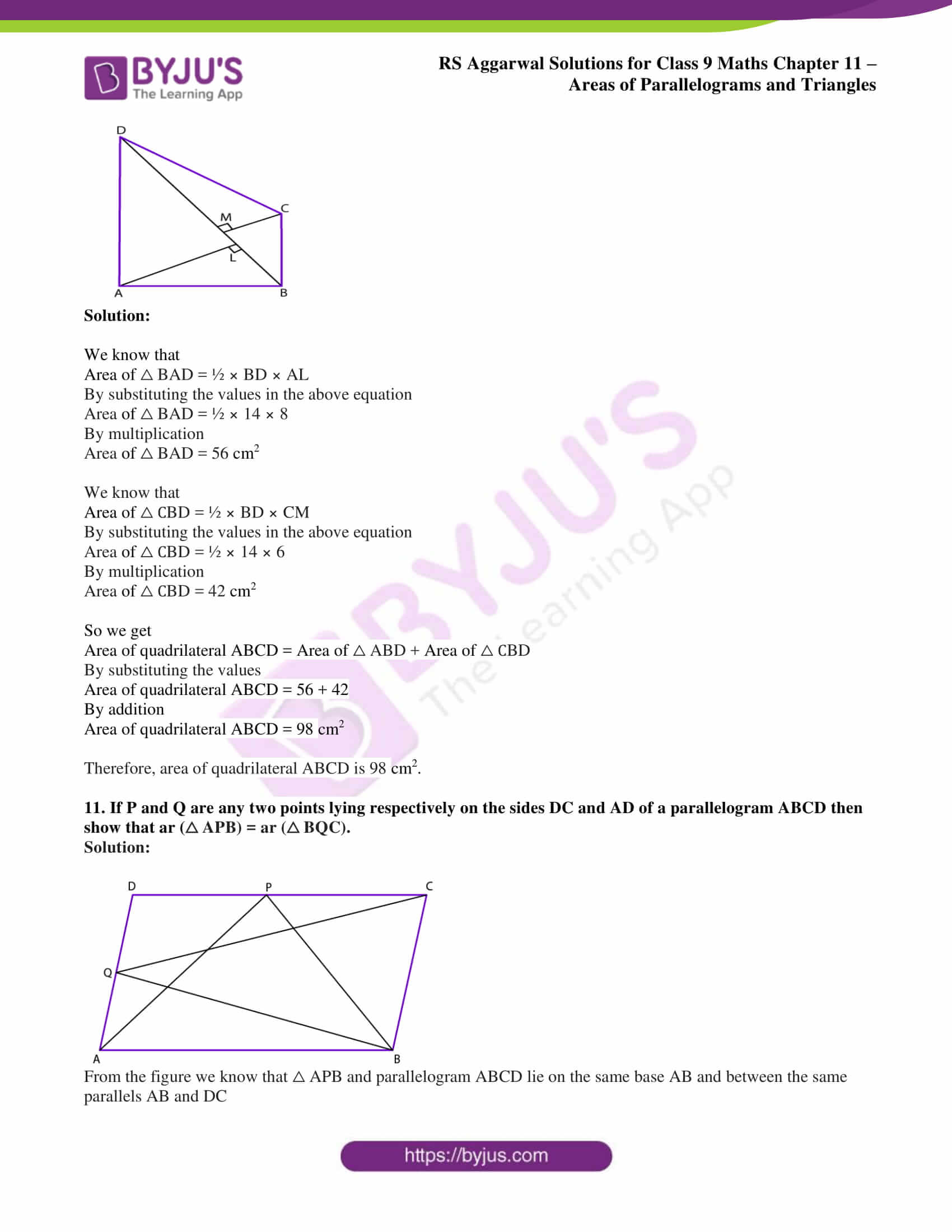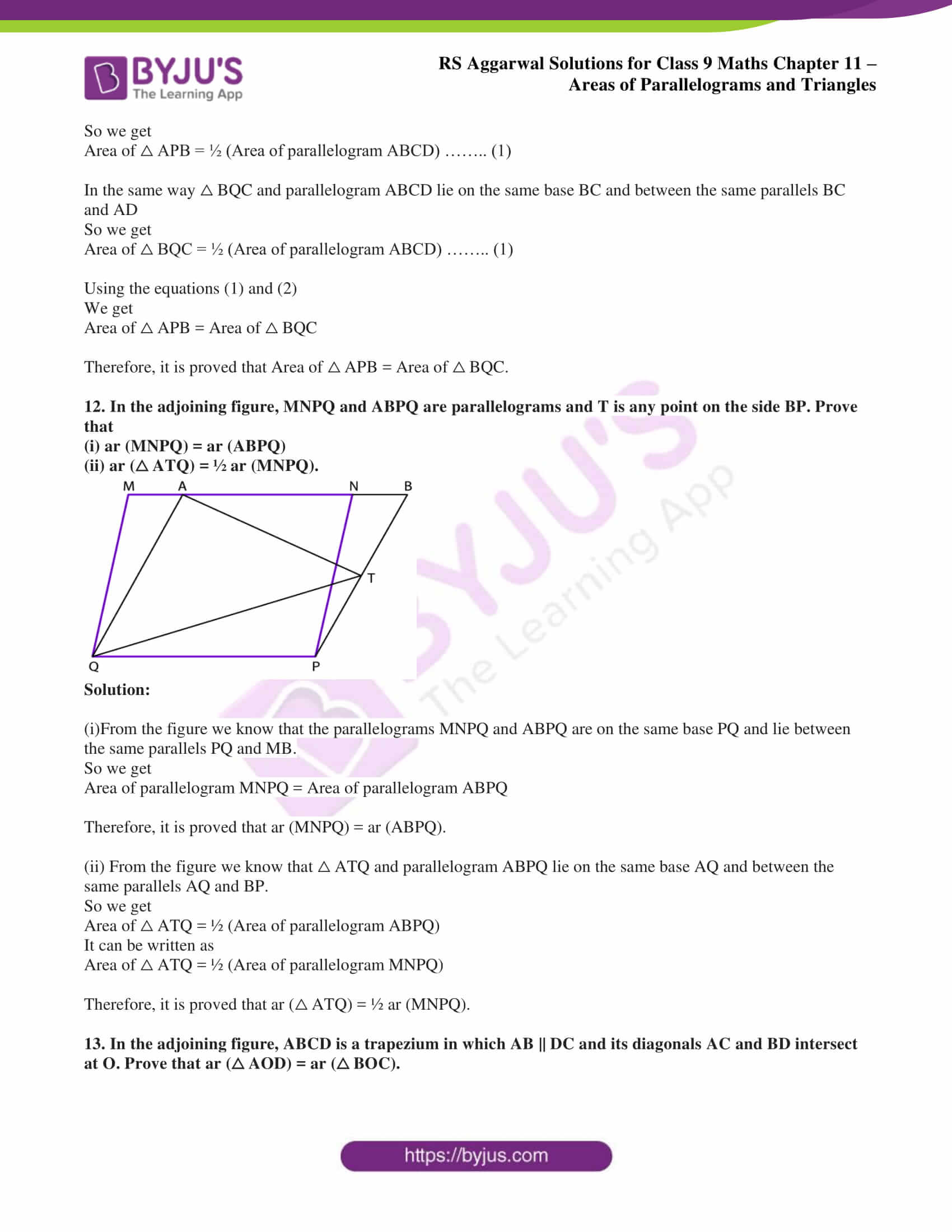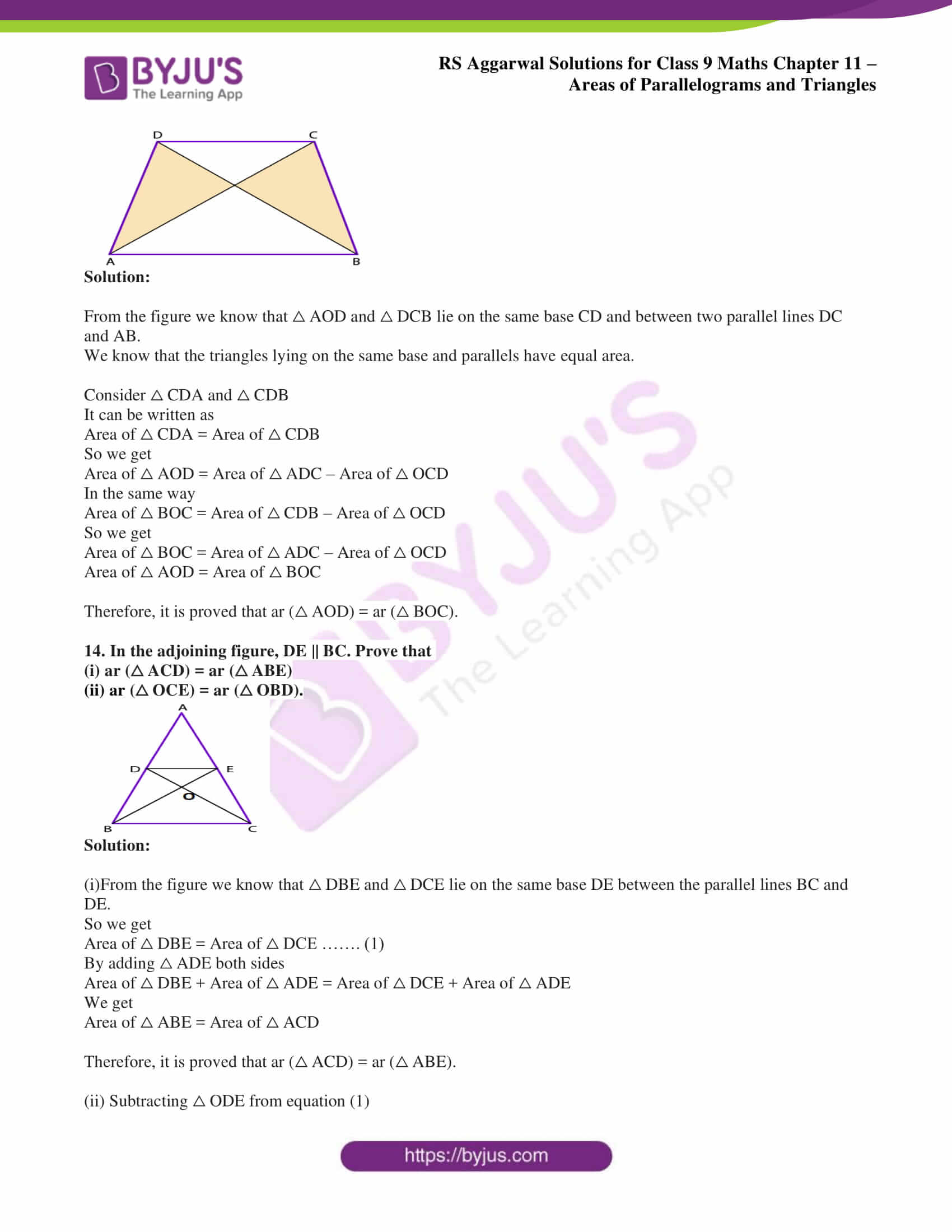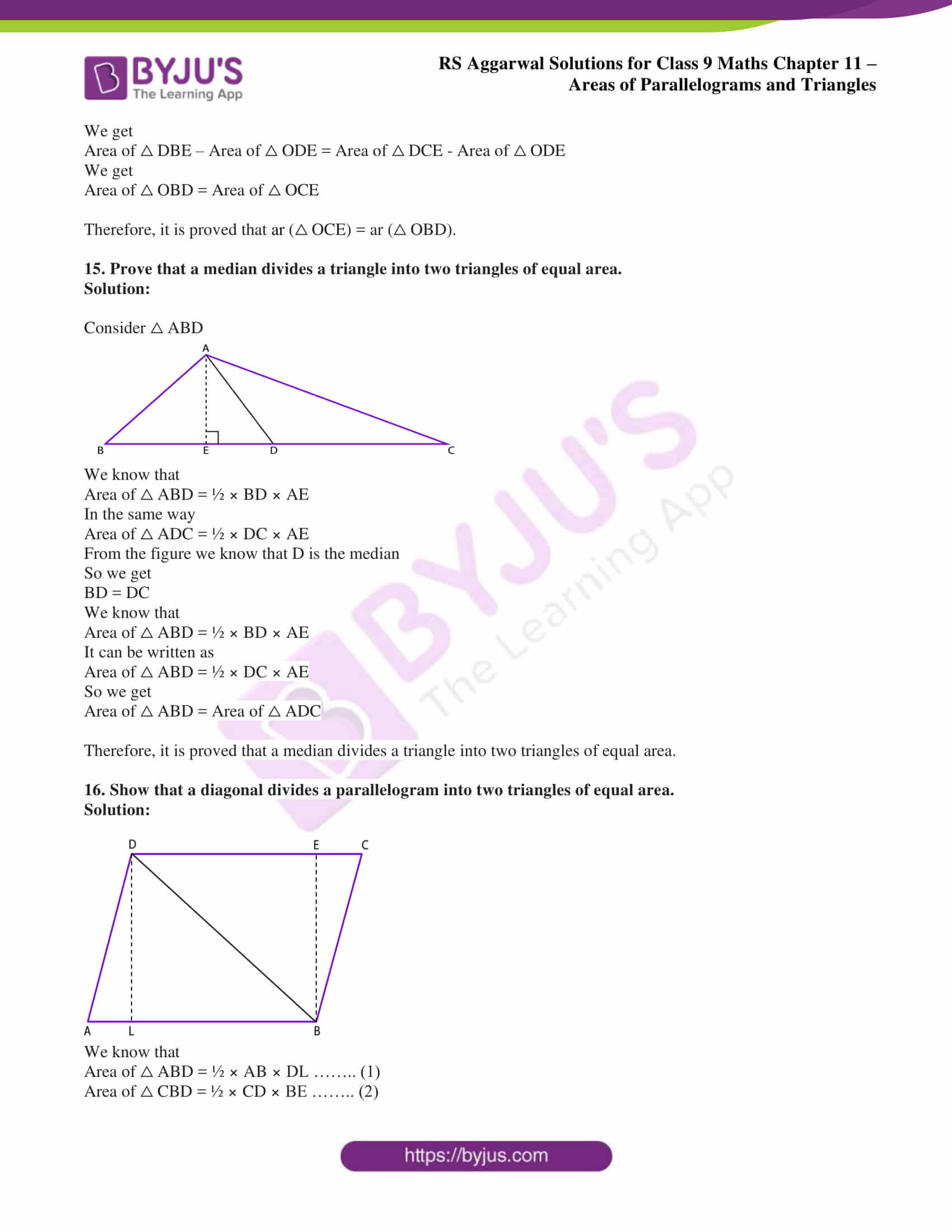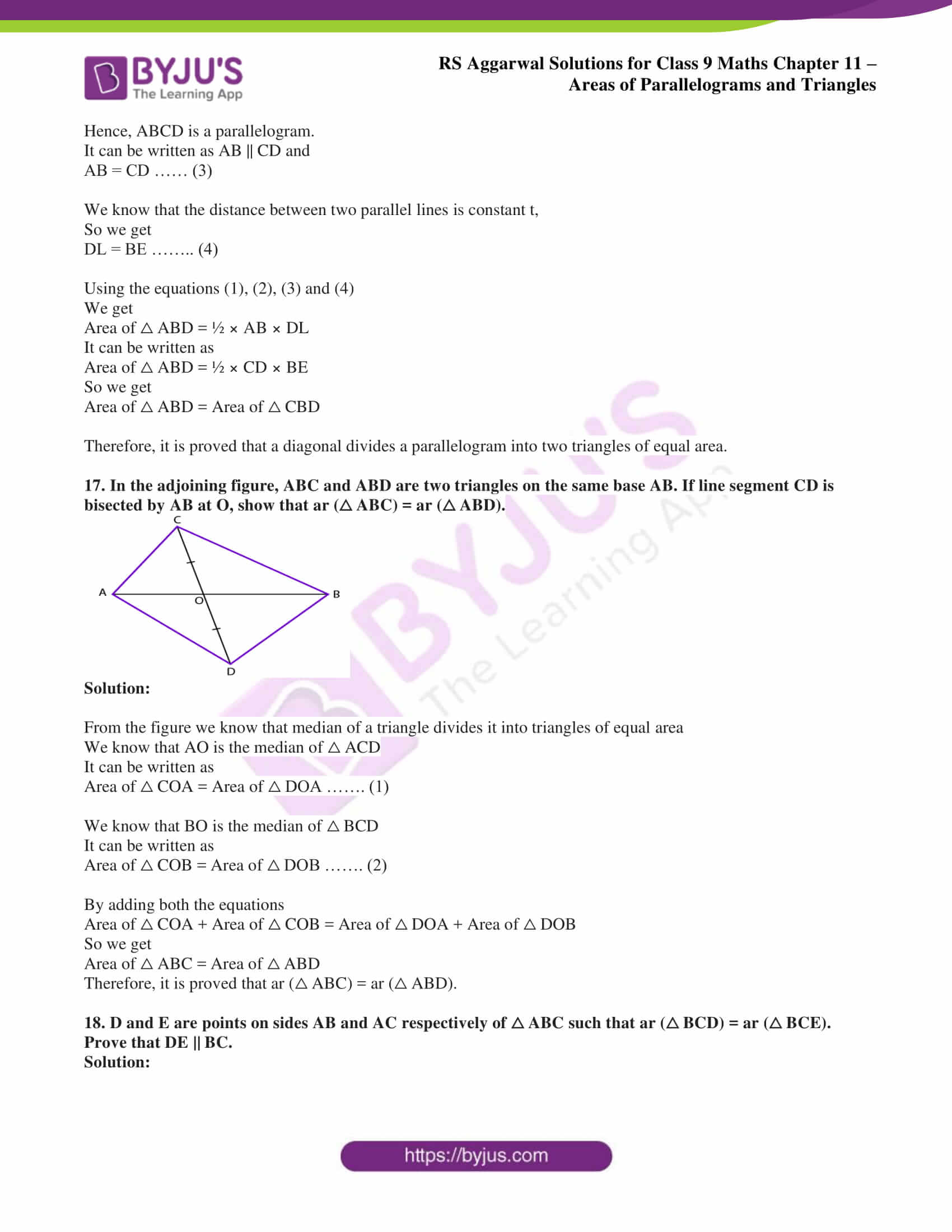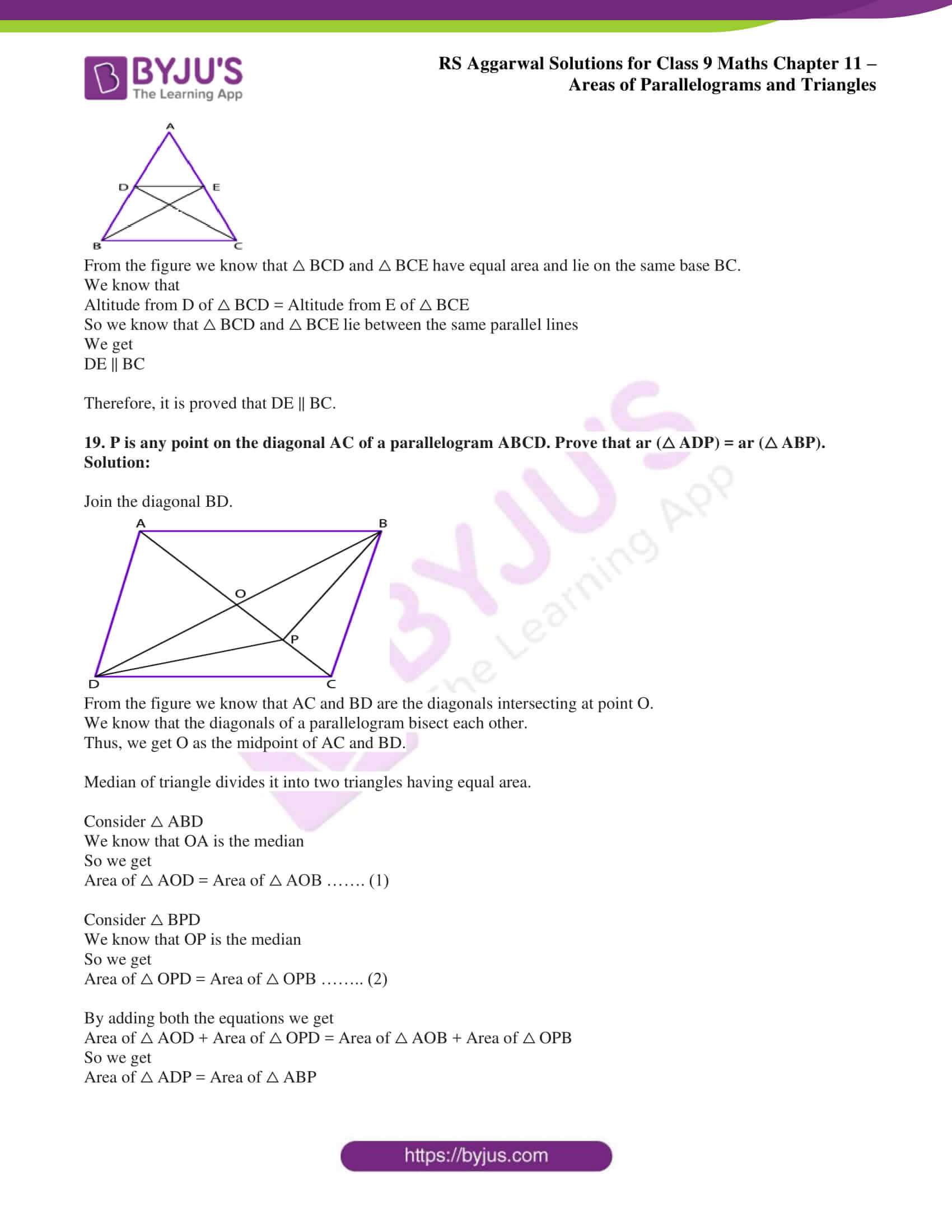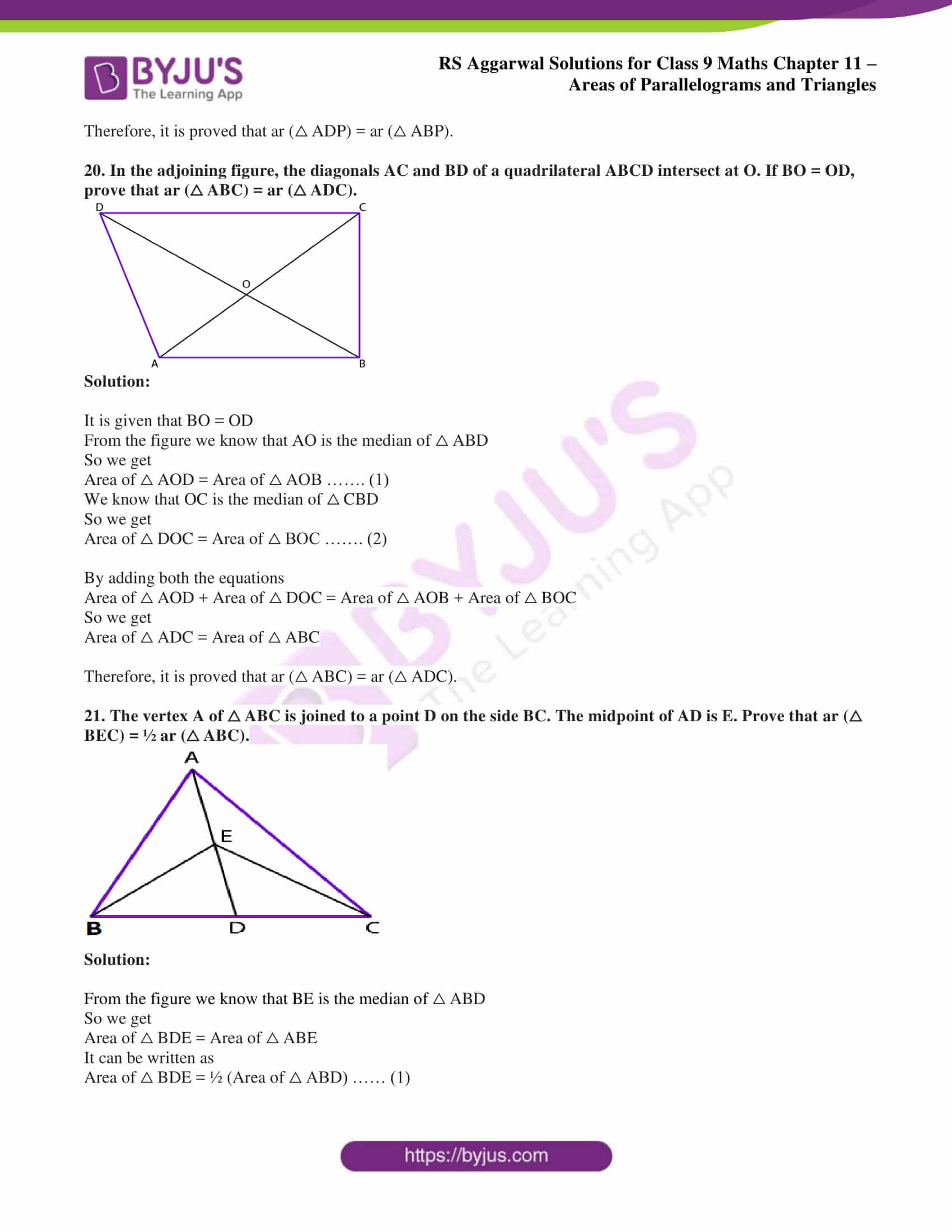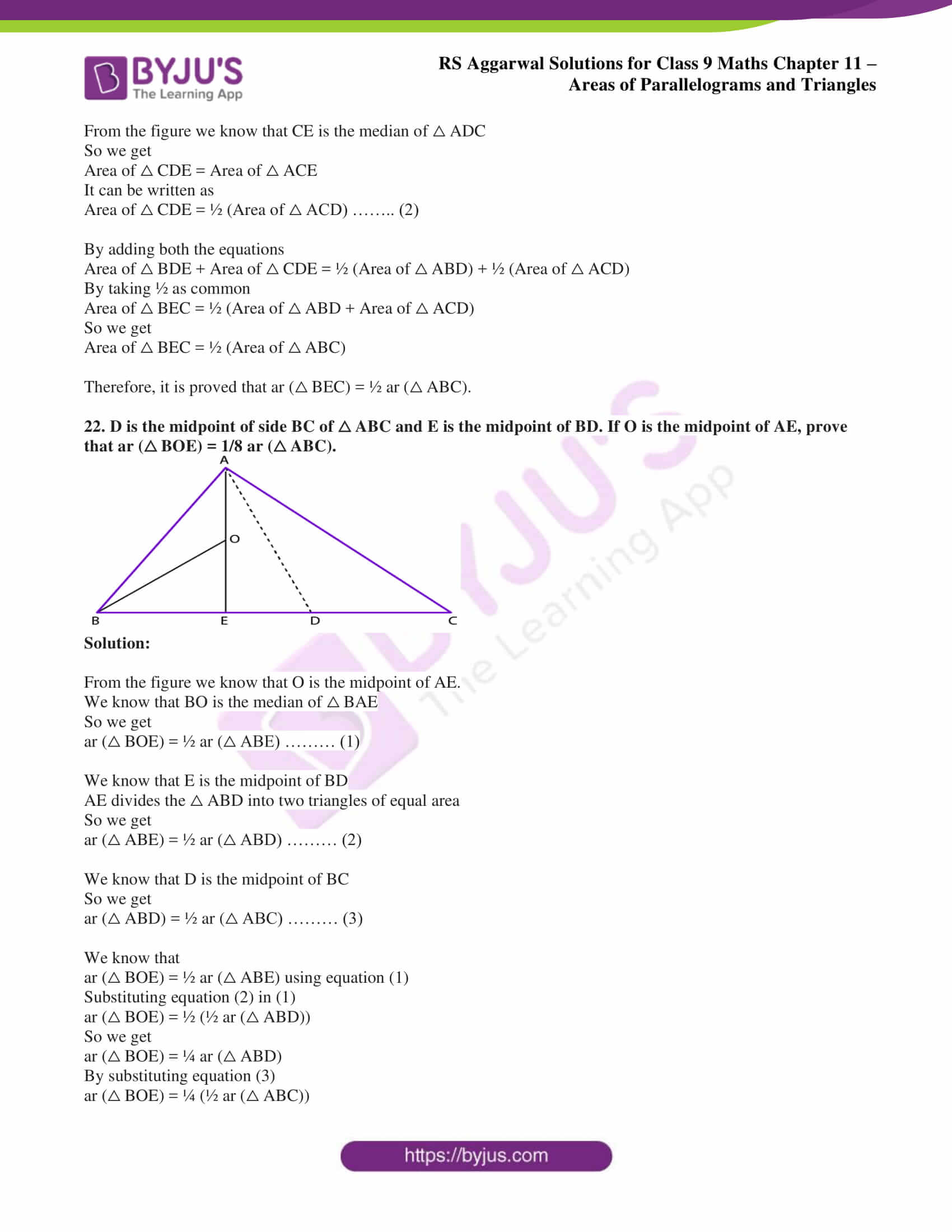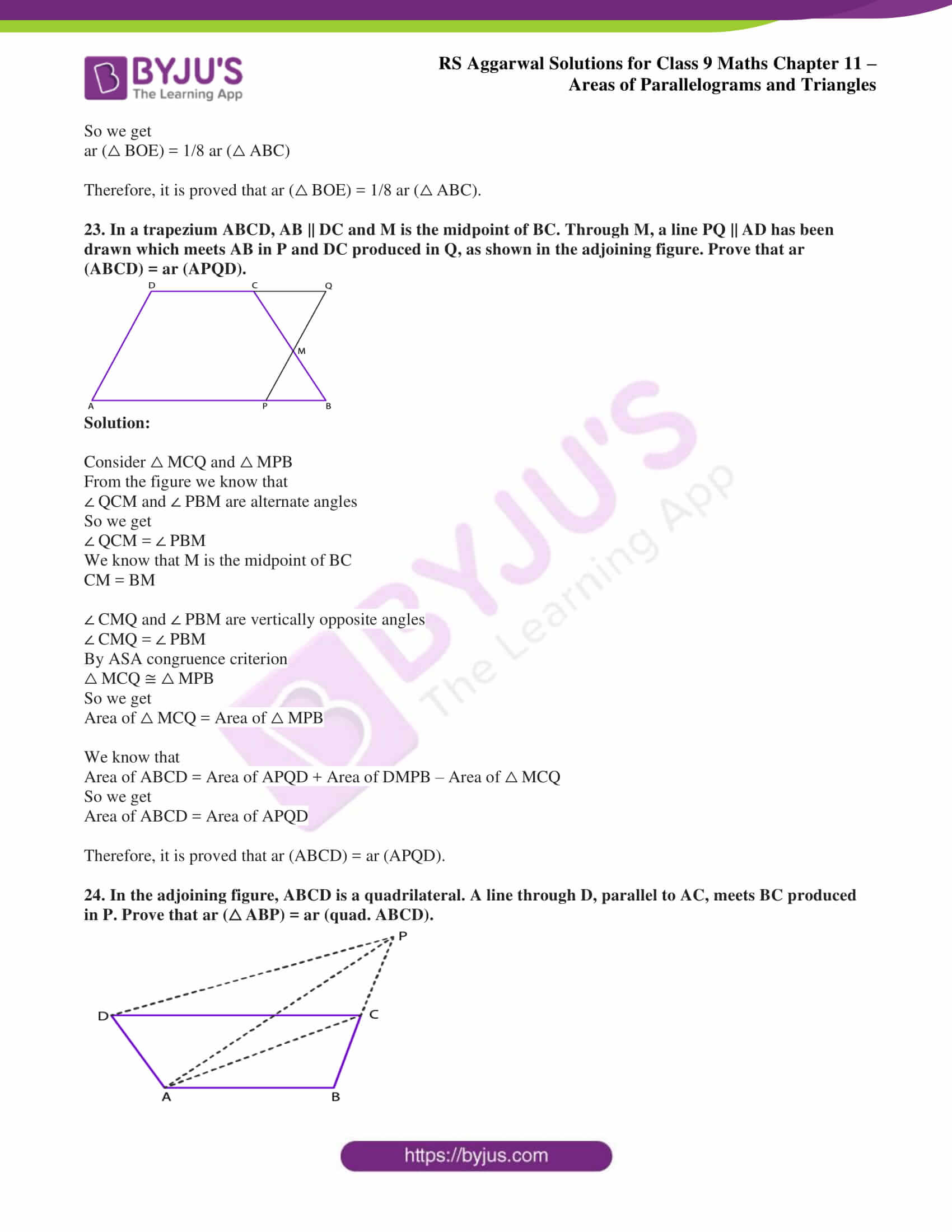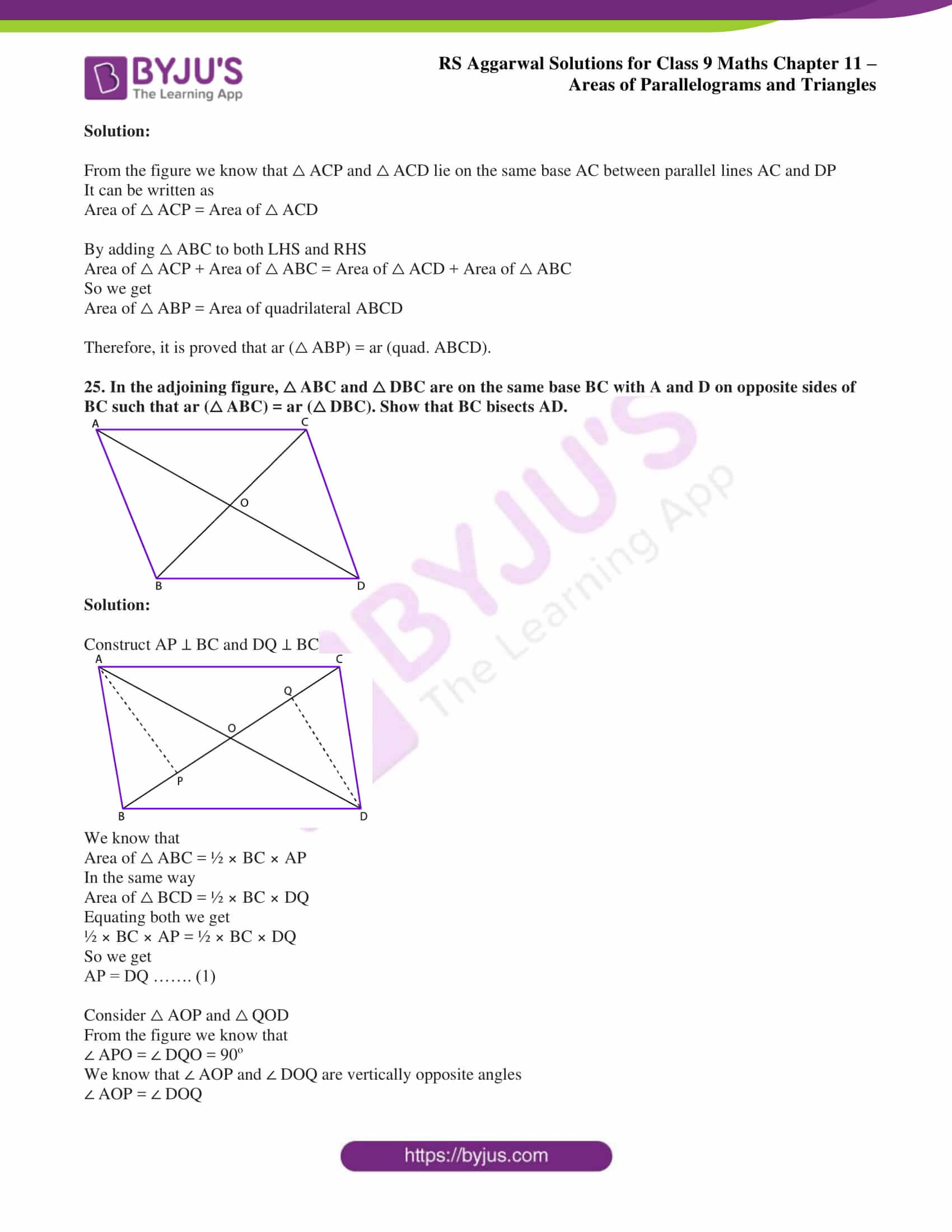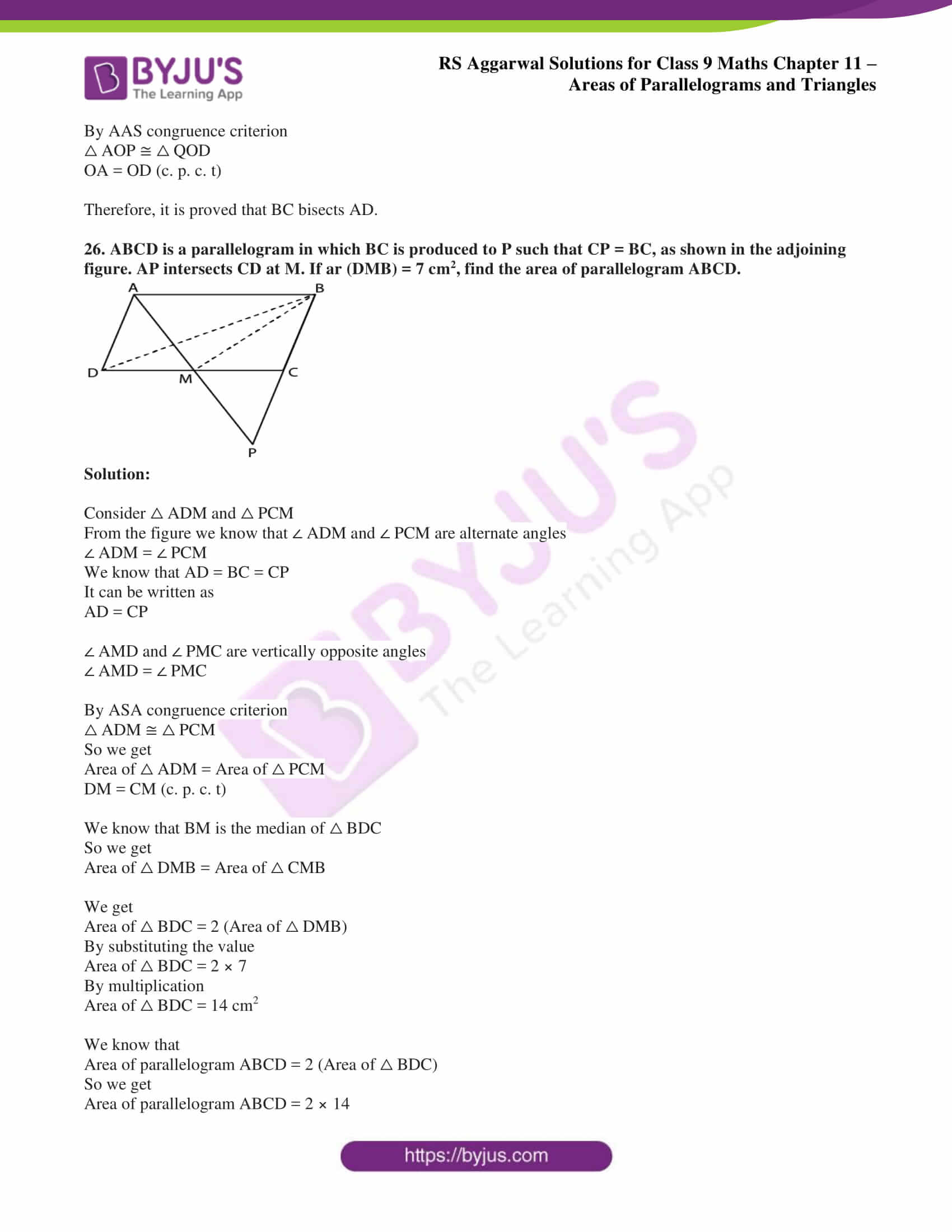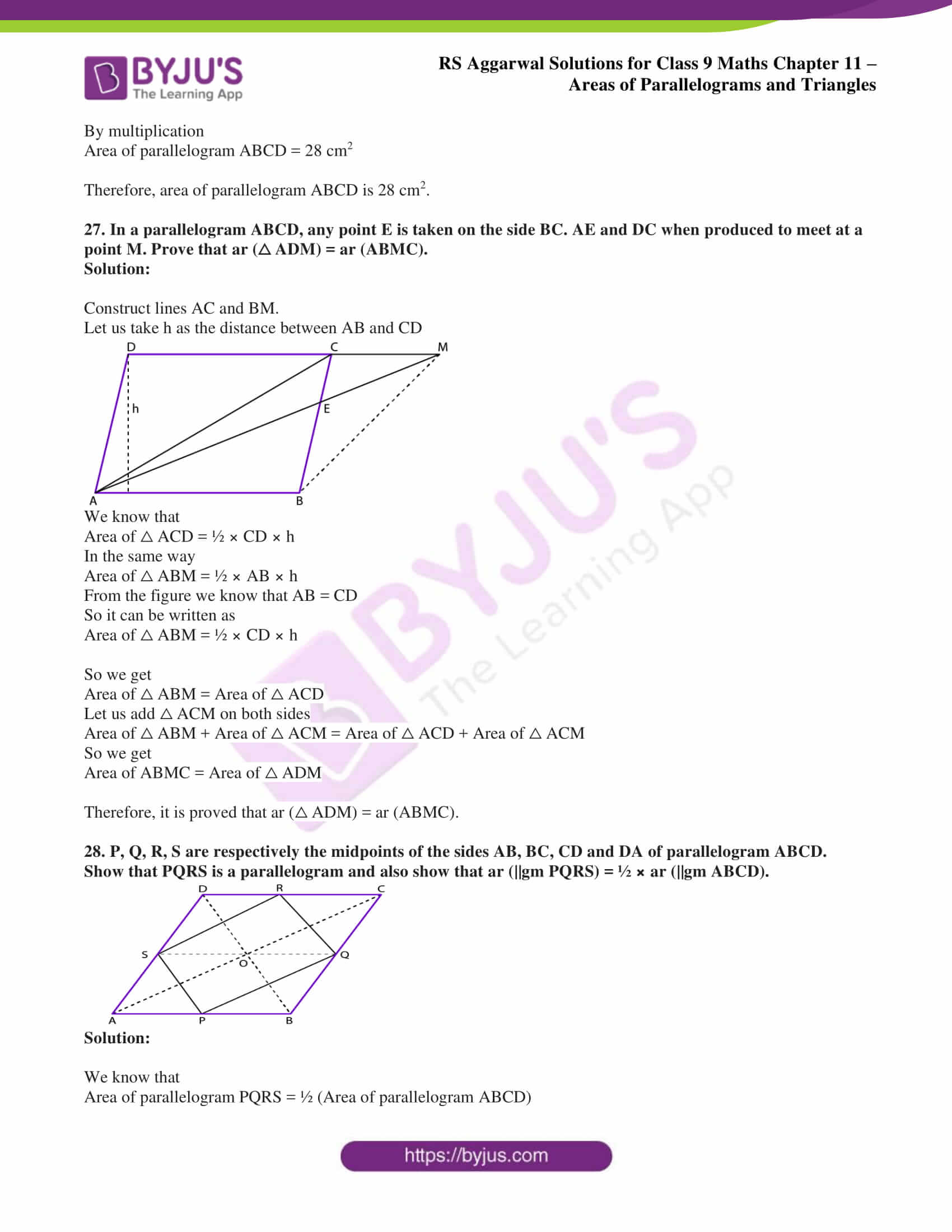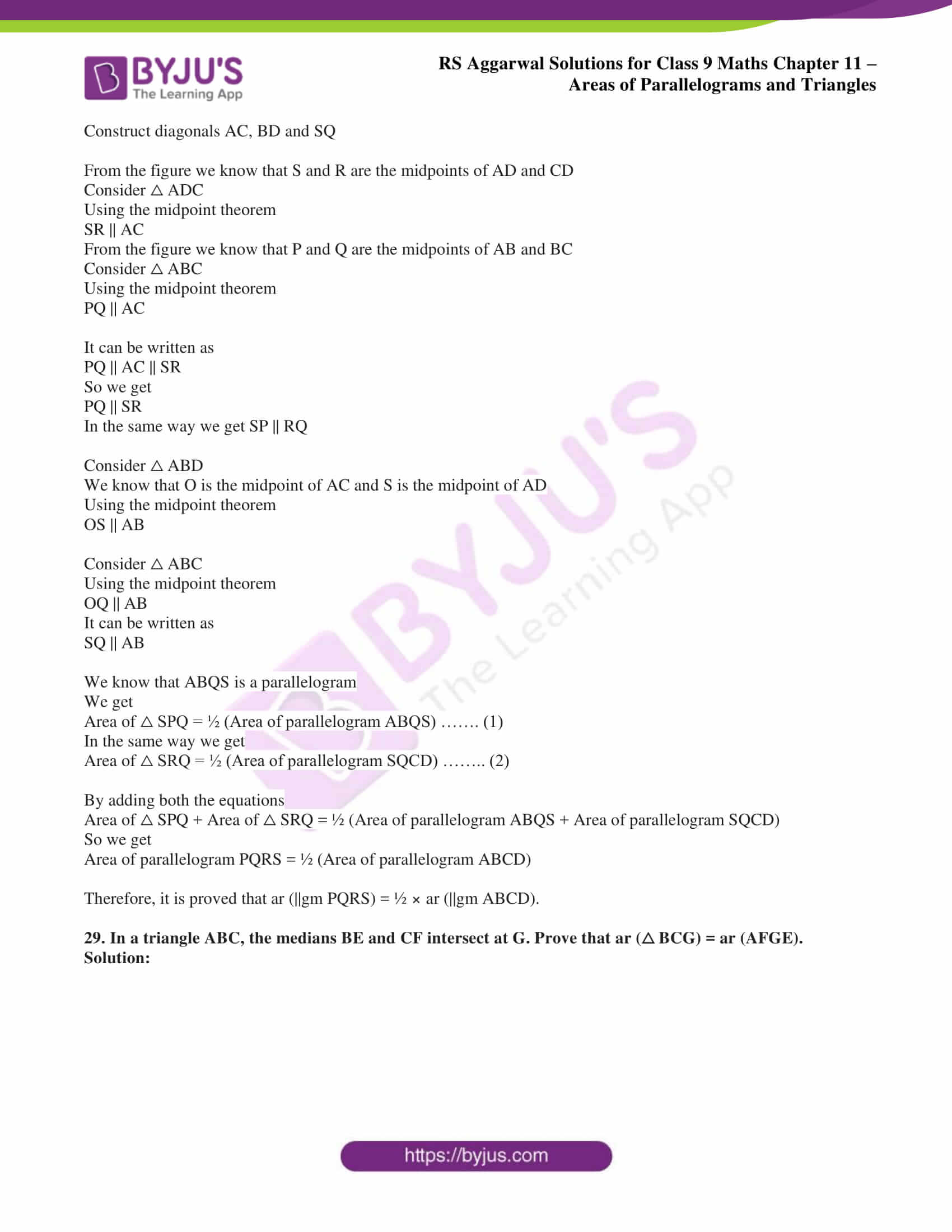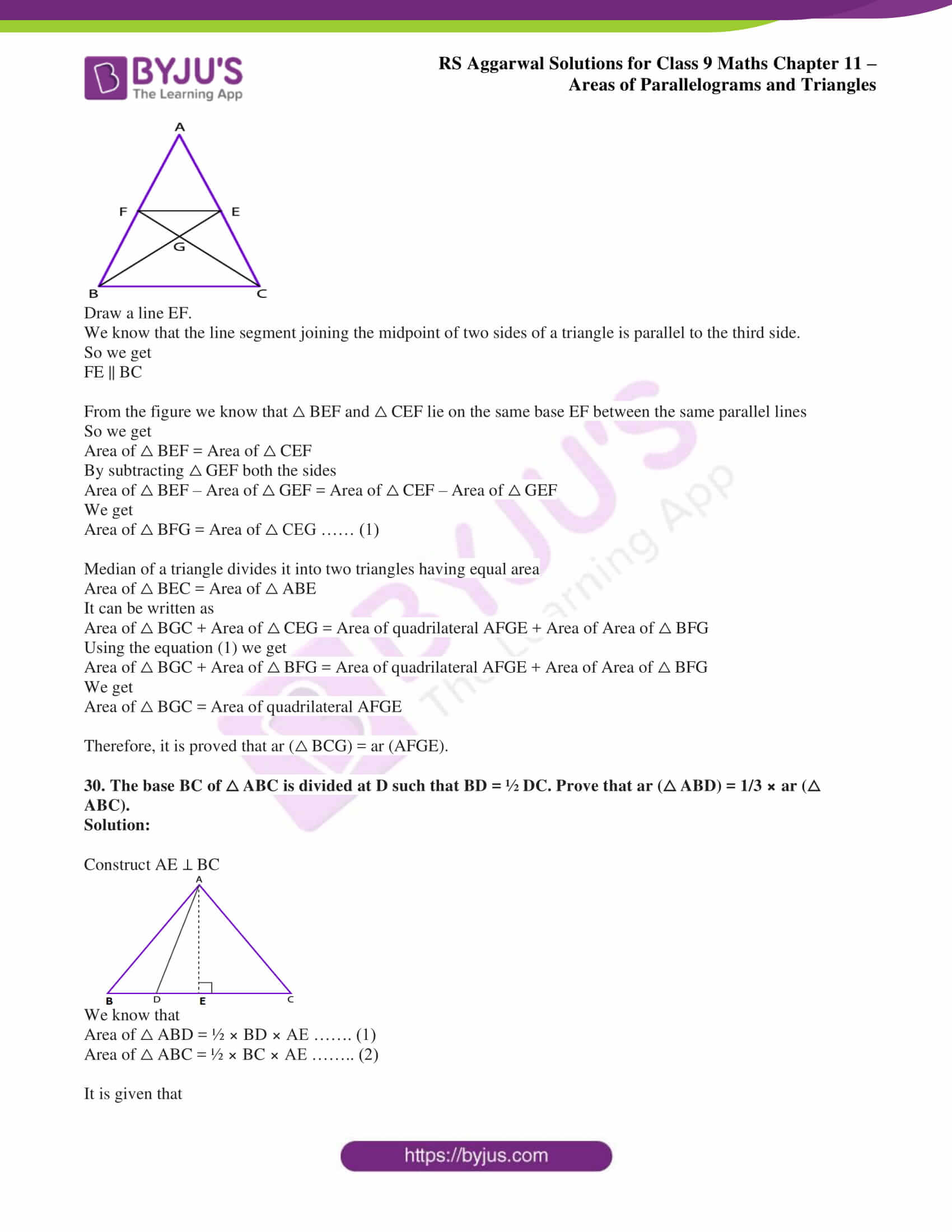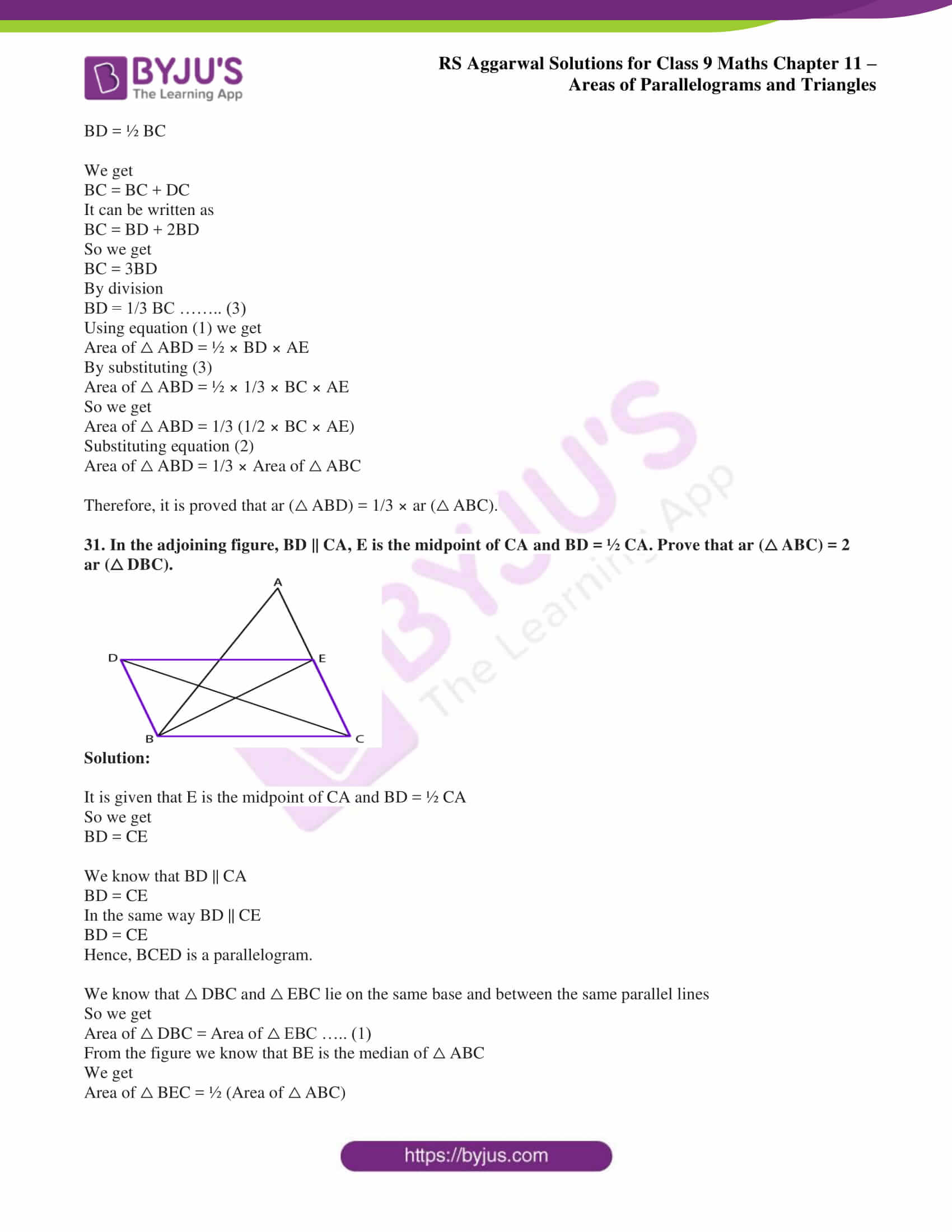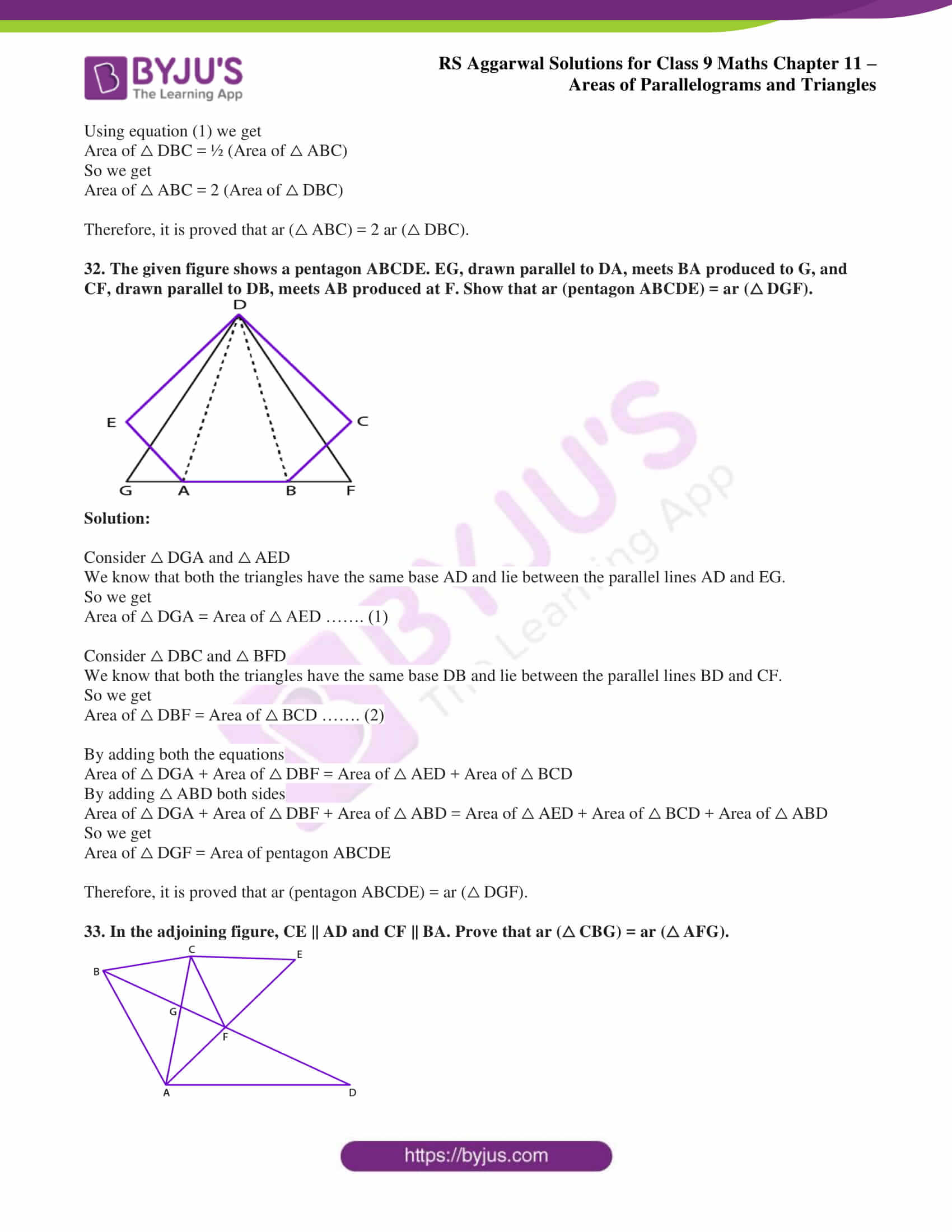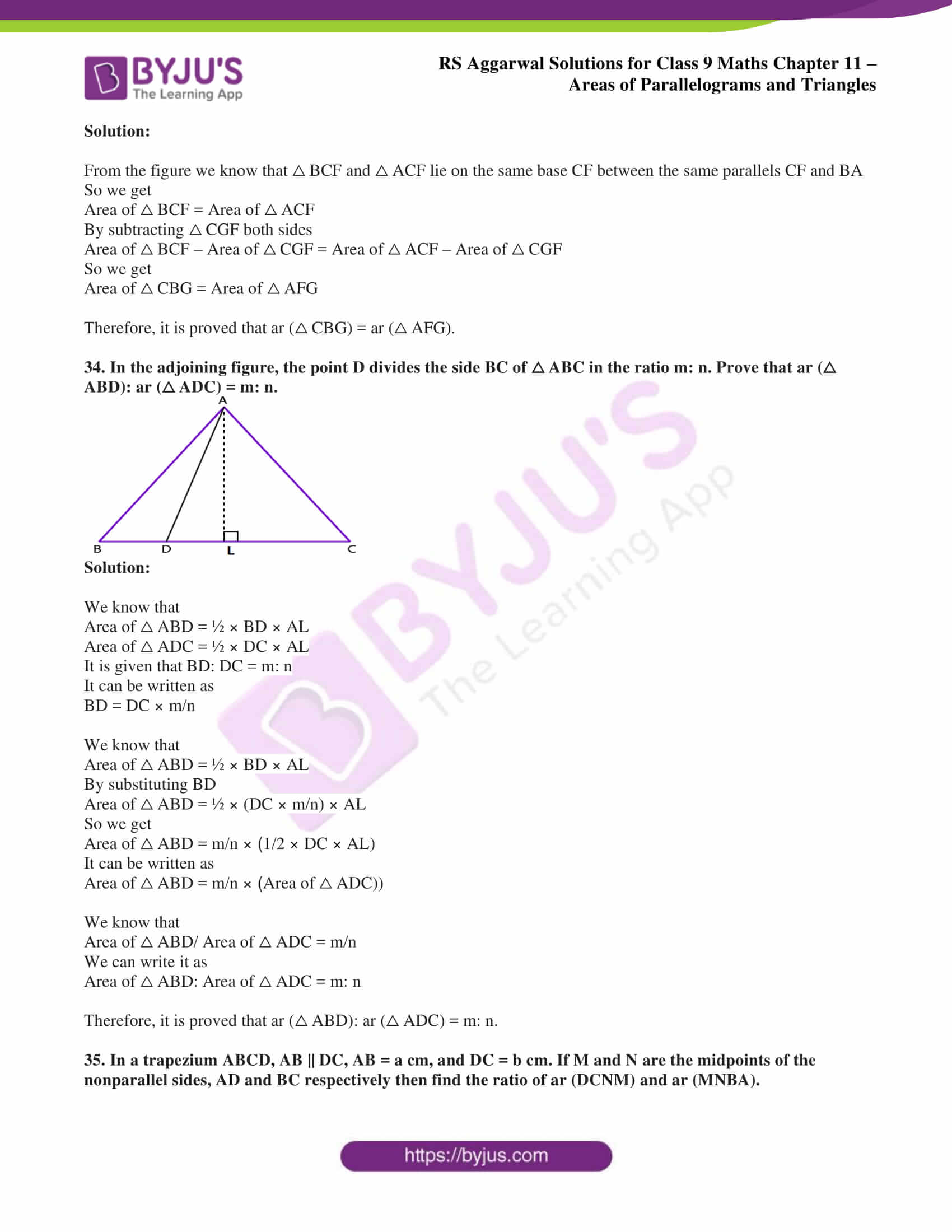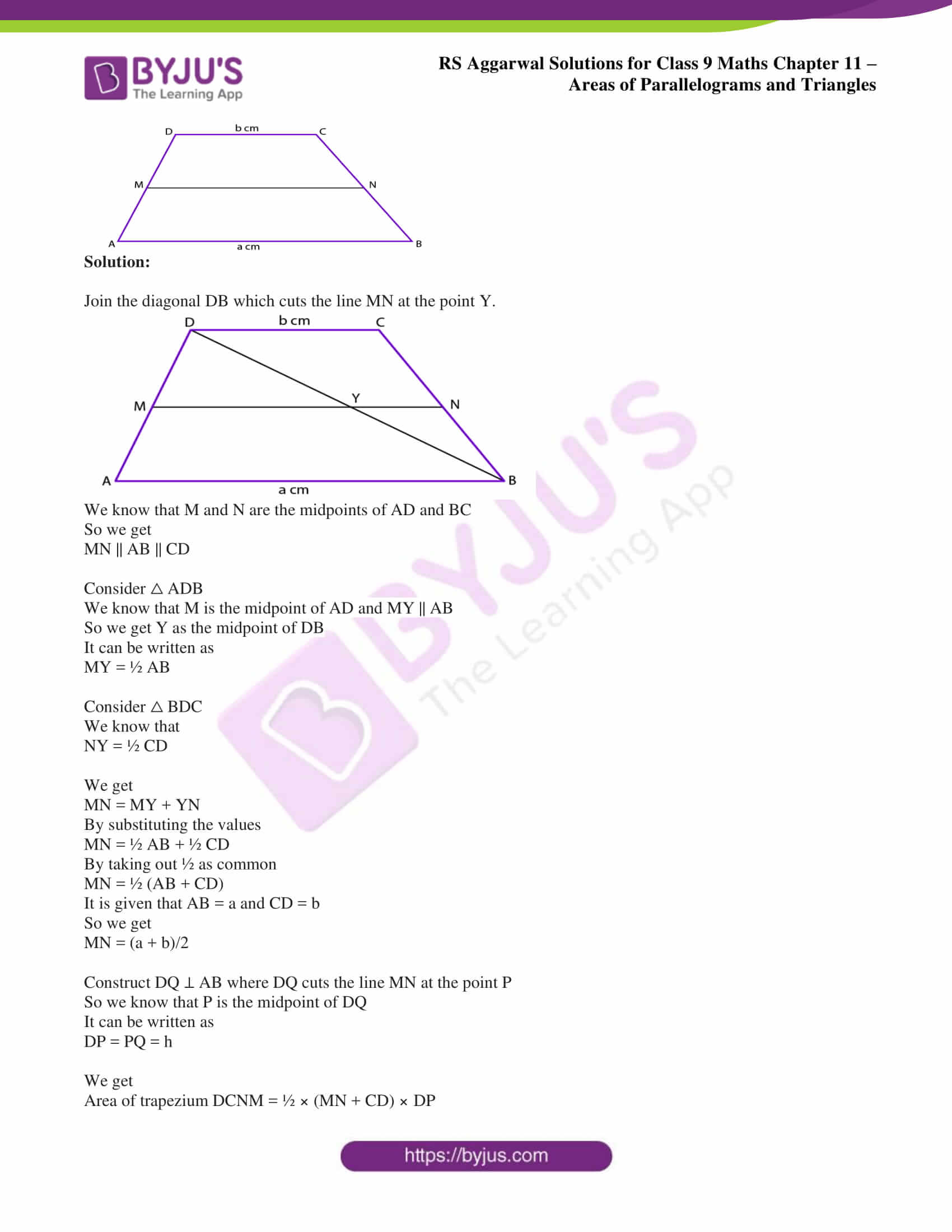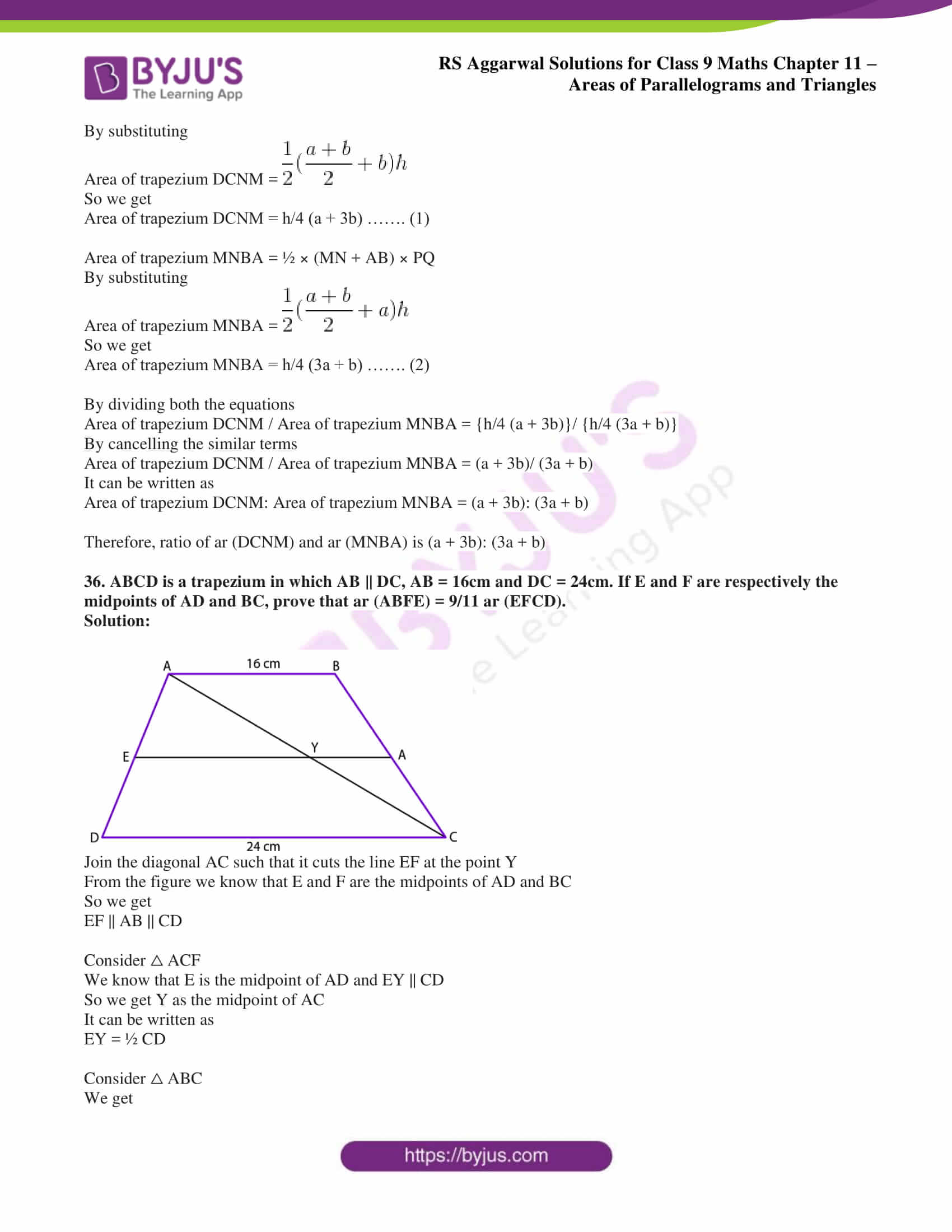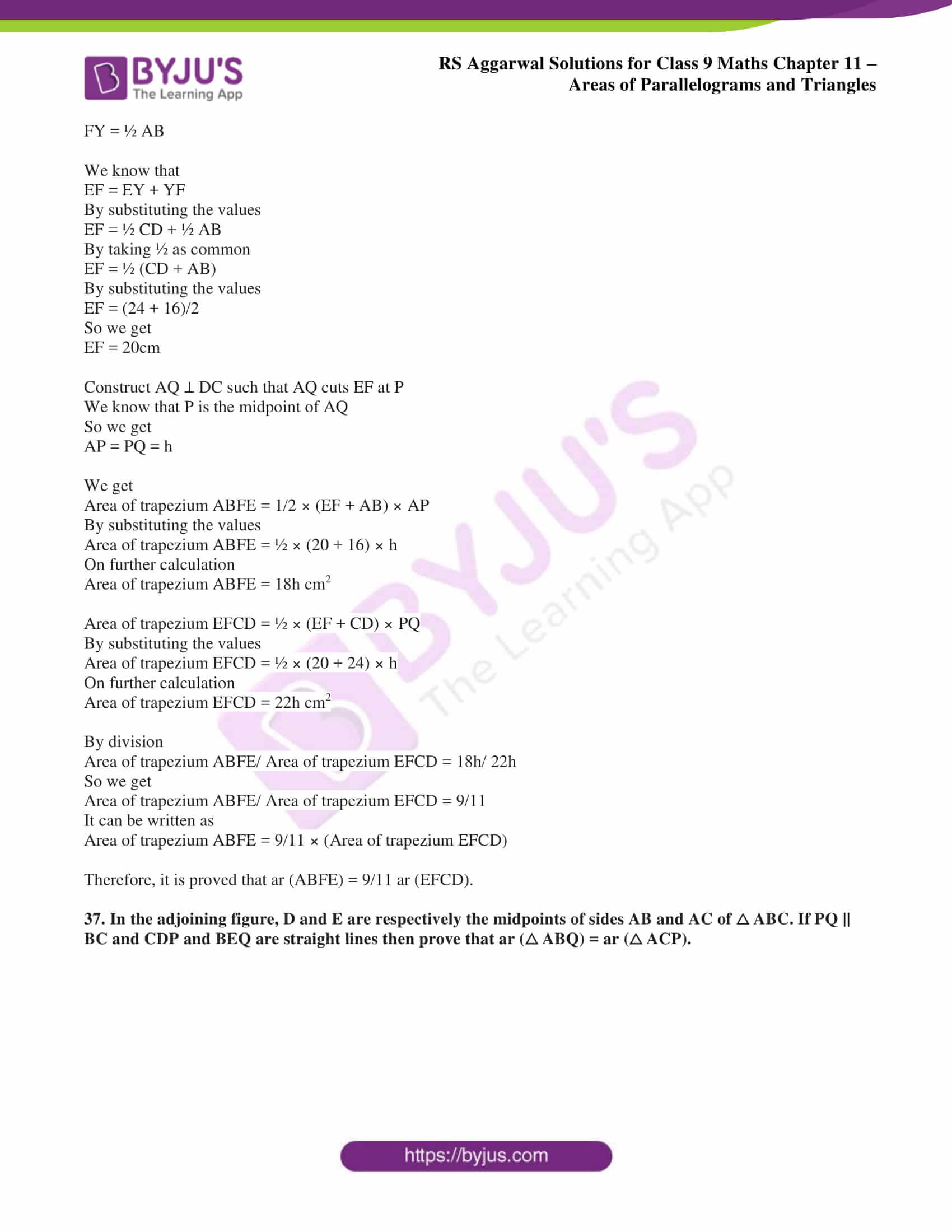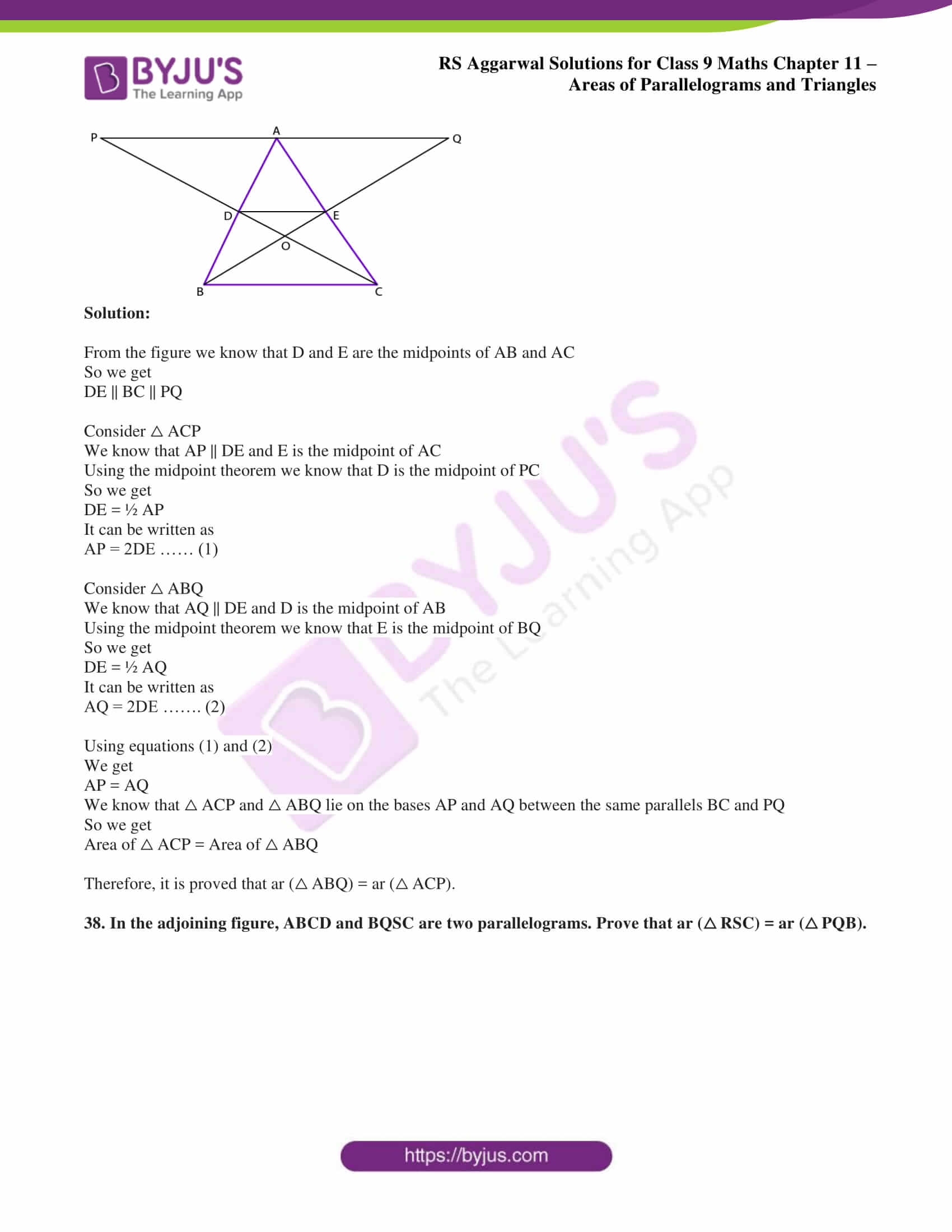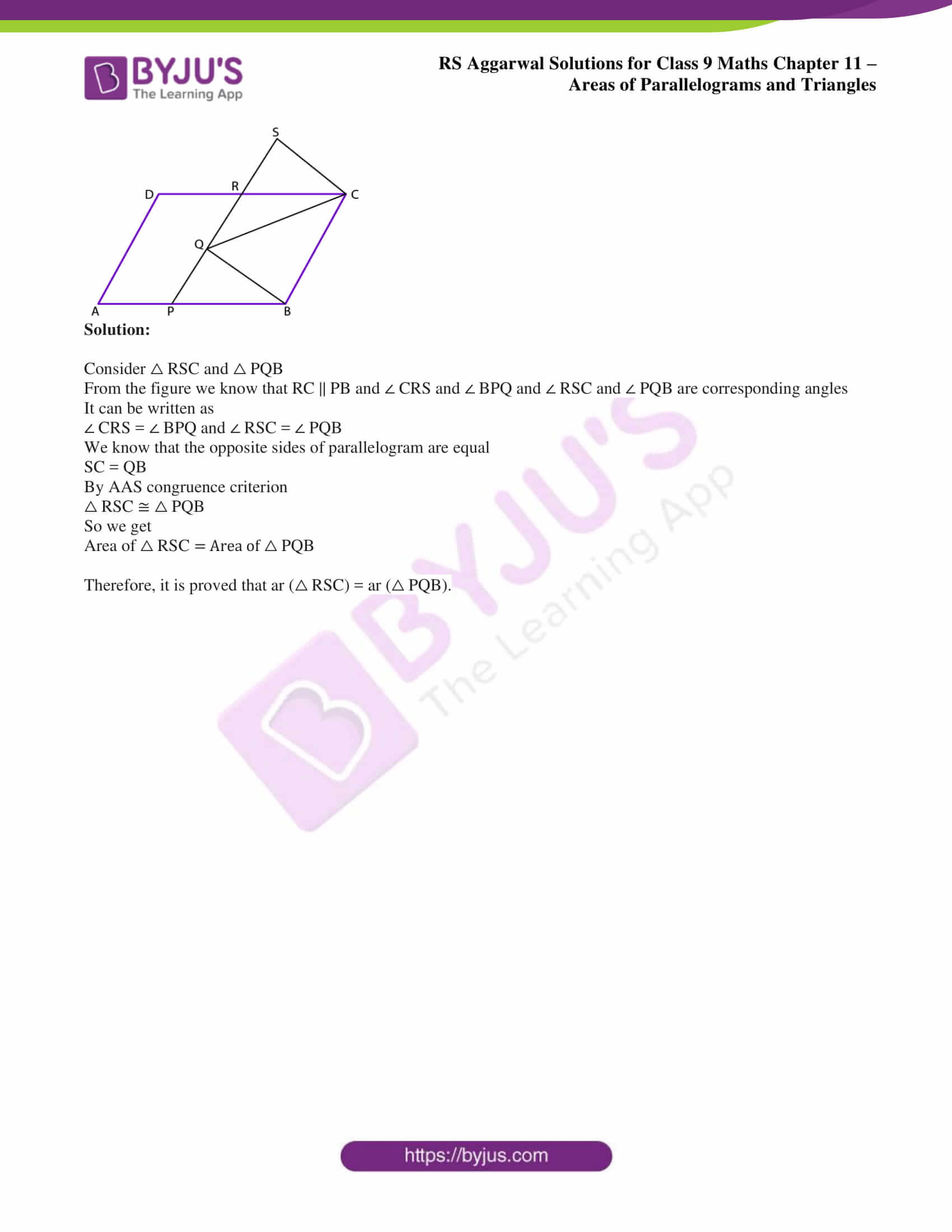## Access RS Aggarwal Solutions for Class 9 Chapter 11: Areas of Parallelograms and Triangles

Exercise 11 page: 387

1. Which of the following figures lie on the same base and between the same parallels. In such a case, write the common base and the two parallels.

(i)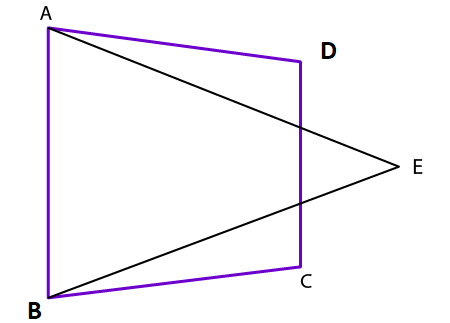(ii)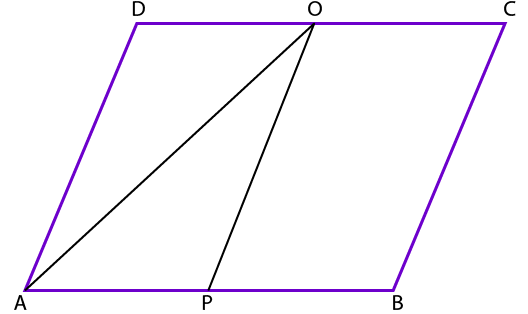(iii)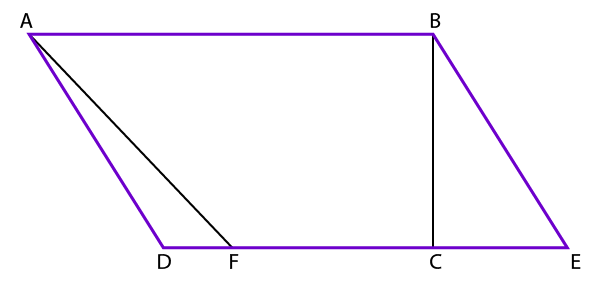(iv)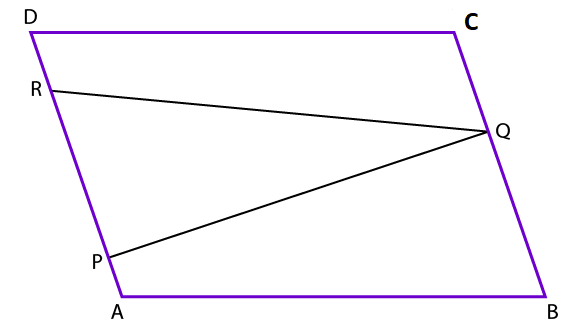(v)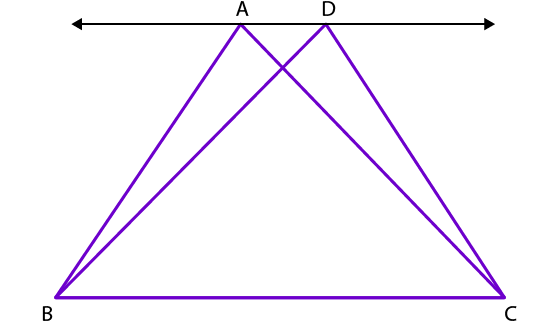(vi)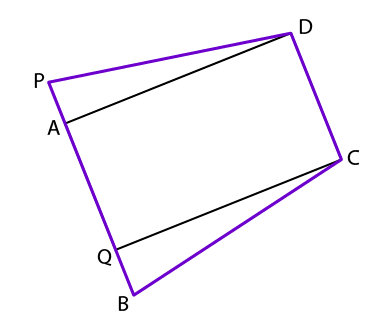Solution:

(i) It does not lie on the same base and between the same parallels.

(ii) It does not lie on the same base and between the same parallels.

(iii) The given figure, lies on the same base and between the same parallels. The common base is AB and the two parallels are AB and DE.

(iv) It does not lie on the same base and between the same parallels.

(v) The given figure, lies on the same base and between the same parallels. The common base is BC and the two parallels are BC and AD.

(vi) The given figure lies on the same base and between the same parallels. The common base is CD and the two parallels are CD and BP.

2. In the adjoining figure, show that ABCD is a parallelogram. Calculate the area of parallelogram ABCD.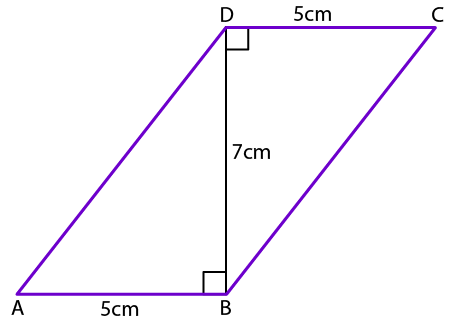Solution:

Construct AM ⊥ DC and CL ⊥ AB. Extend the lines DC and AB and join AC.

We know that

Area of the quadrilateral ABCD = area of triangle ABD + area of triangle DCB

From the figure we know that

Area of △ ABD = Area of △ DCB

So it can be written as

Area of the quadrilateral ABCD = 2 (Area of △ ABD)

We get

Area of △ ABD = ½ (area of quadrilateral ABCD) ……… (1)

Area of quadrilateral ABCD = area of △ ABC + area of △ CDA

From the figure we know that

Area of △ ABC = Area of △ CDA

So it can be written as

Area of the quadrilateral ABCD = 2 (Area of △ ABC)

We get

Area of △ ABC = ½ (area of quadrilateral ABCD) ……… (2)

Using the equations (1) and (2)

Area of △ ABD = Area of △ ABC = ½ BD = ½ CL

It can be written as

CL = BD

In the same way we get

DC = AB and AD = BC

Hence, ABCD is a parallelogram

Area of parallelogram ABCD = base × height

= 5 × 7

= 35 cm2

Therefore, area of parallelogram ABCD is 35 cm2.

3. In a parallelogram ABCD, it is being given that AB = 10cm and the altitudes corresponding to the sides AB and AD are DL = 6cm and BM = 8cm, respectively. Find AD.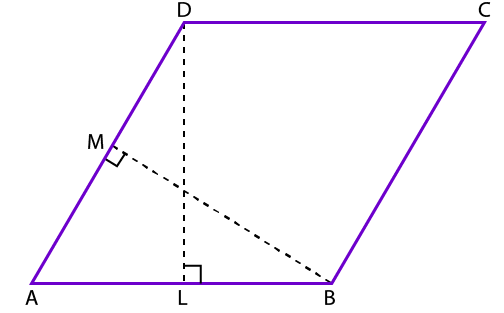Solution:

From the figure we know that

Area of the parallelogram ABCD = base ×height

So we get

AB × DL = AD × BM

By substituting the values in the above equation

10 × 6 = AD × BM

It is given that BM = 8cm

AD × BM = 60 cm2

By division

So we get

4. Find the area of a figure formed by joining the midpoints of the adjacent sides of a rhombus with diagonals 12 cm and 16 cm.

Solution:

We know that ABCD is a rhombus with P, Q, R and S as the midpoints of AB, BC, CD and DA.

AC and BD diagonals are joined.

Using the midpoint theorem

We know that

PQ = ½ AC

By substituting the values we get

PQ = ½ (16)

By division

PQ = 8cm

In △ DAC we know that S and R are the midpoints of AD and DC

Using the midpoint theorem

SR = ½ AC

By substituting the values

SR = ½ (12)

By division

SR = 6cm

Consider the rectangle PQRS

Area of the rectangle PQRS = length × breadth

So we get

Area of the rectangle PQRS = 6 × 8

By multiplication

Area of the rectangle PQRS = 48 cm2

Therefore, area of the figure is 48 cm2.

5. Find the area of a trapezium whose parallel sides are 9cm and 6cm respectively and the distance between these sides is 8cm.

Solution: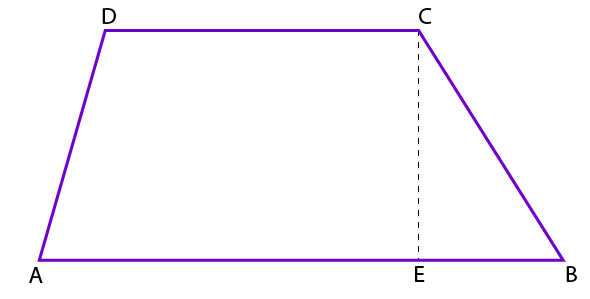We know that

Area of trapezium = ½ (sum of parallel sides × distance between them)

By substituting the values

Area of trapezium = ½ ((9 + 6) × 8)

On further calculation

Area of trapezium = ½ (120)

So we get

Area of trapezium = 60 cm2

Therefore, area of the trapezium is 60 cm2.

6. (i) Calculate the area of quad. ABCD, given in Fig. (i).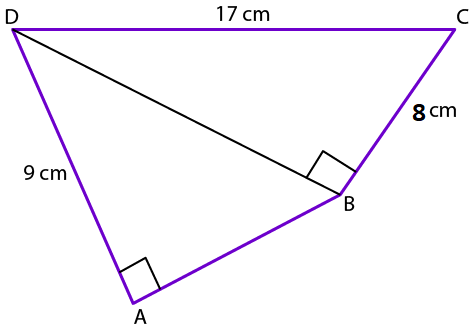(ii) Calculate the area of trap. PQRS, given in Fig. (ii).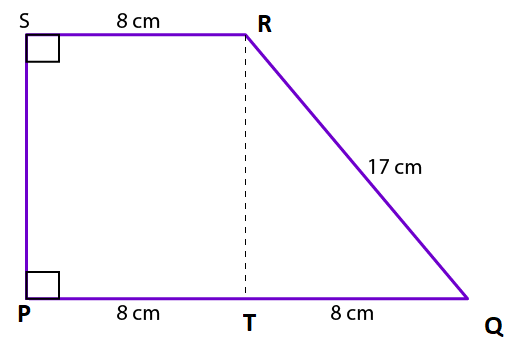Solution:

(i)Consider △ BCD

Using the Pythagoras theorem

We can write it as

DB2 + BC2 = DC2

By substituting the values

DB2 + 82 = 172

By subtraction

DB2 = 172 – 82

DB2 = 289 – 64

By subtraction

DB2 = 225

By taking the square root

DB = √ 225

So we get

DB = 15cm

We can find

Area of △ BCD = ½ × b × h

By substituting the values

Area of △ BCD = ½ × 8 × 15

On further calculation

Area of △ BCD = 60 cm2

Using the Pythagoras theorem

We can write it as

DA2 + AB2 = DB2

By substituting the values

AB2 + 92 = 152

By subtraction

AB2 = 152 – 92

AB2 = 225 – 81

By subtraction

AB2 = 144

By taking the square root

AB = √ 144

So we get

AB = 12cm

We can find

Area of △ DAB = ½ × b × h

By substituting the values

Area of △ DAB = ½ × 9 × 12

On further calculation

Area of △ DAB = 54 cm2

So we get

Area of quadrilateral ABCD = area of △ DAB + area of △ BCD

By substituting the values

Area of quadrilateral ABCD = 54 + 60

Area of quadrilateral ABCD = 114 cm2

Therefore, the area of quadrilateral ABCD is 114 cm2.

(ii) From the figure

Using the Pythagoras theorem in △ RTQ

We get

RT2 + TQ2 = RQ2

By substituting the values

RT2 + 82 = 172

On further calculation

RT2 = 172 – 82

So we get

RT2 = 289 – 64

By subtraction

RT2 = 225

By taking square root

RT = √ 225

RT = 15cm

We can find the area of trapezium

Area of trapezium PQRS = ½ (sum of parallel sides × distance between them)

So we get

Area of trapezium PQRS = ½ ((8 + 16) × 15)

On further calculation we get

Area of trapezium PQRS = 180 cm2

Therefore, the area of trapezium PQRS is 180 cm2.

7. In the adjoining figure, ABCD is a trapezium in which AB || DC; AB = 7cm; AD = BC = 5cm and the distance between AB and DC is 4cm. Find the length of DC and hence, find the area of trap. ABCD.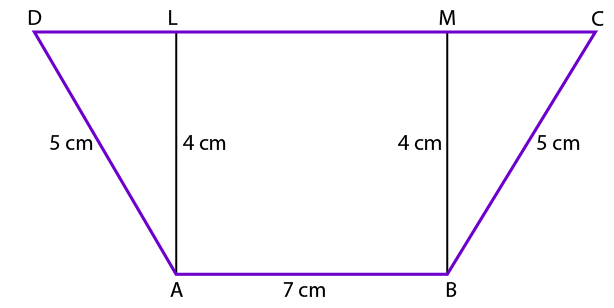Solution:

Consider △ ALD

Based on the Pythagoras theorem

By substituting the values

42 + DL2 = 52

So we get

DL2 = 52 – 42

DL2 = 25 – 16

By subtraction

DL2 = 9

By taking square root

DL = √ 9

So we get

DL = 3 cm

Consider △ BMC

Based on the Pythagoras theorem

MC2 + MB2 = CB2

By substituting the values

MC2 + 42 = 52

So we get

MC2 = 52 – 42

MC2 = 25 – 16

By subtraction

MC2 = 9

By taking square root

MC = √ 9

So we get

MC = 3 cm

From the figure we know that LM = AB = 7cm

So we know that CD = DL + LM + MC

By substituting the values

CD = 3 + 7 + 3

CD = 13cm

Area of Trapezium ABCD = ½ (sum of parallel sides × distance between them)

So we get

Area of Trapezium ABCD = ½ × (CD + AB) × AL

By substituting the values

Area of Trapezium ABCD = ½ × (13 + 7) × 4

On further calculation

Area of Trapezium ABCD = 20 × 2

By multiplication

Area of Trapezium ABCD = 40 cm2

Therefore, length of DC = 13cm and area of trapezium ABCD = 40 cm2.

8. BD is one of the diagonals of a quad. ABCD. If AL ⊥ BD and CM ⊥ BD, show that

Ar (quad. ABCD) = ½ × BD × (AL + CM).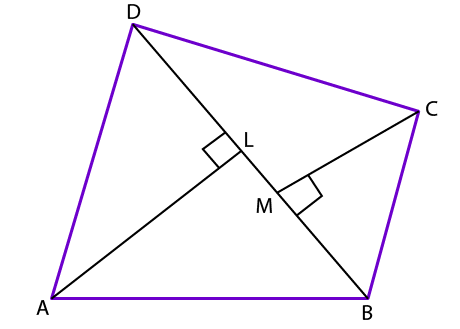Solution:

From the figure we know that

Area of △ ABD = ½ × b × h

It can be written as

Area of △ ABD = ½ × BD × AL

Area of △ CBD = ½ × b × h

It can be written as

Area of △ CBD = ½ × BD × CM

We know that

Area of quadrilateral ABCD = Area of △ ABD + Area of △ CBD

So we get

Area of quadrilateral ABCD = ½ × BD × AL + ½ × BD × CM

It can be written as

Area of quadrilateral ABCD = ½ × BD (AL + CM)

Therefore, it is proved that Area of quadrilateral ABCD = ½ × BD (AL + CM).

9. M is the midpoint of the side AB of a parallelogram ABCD. If ar (AMCD) = 24 cm2, find ar (△ ABC).

Solution:

Join the diagonal AC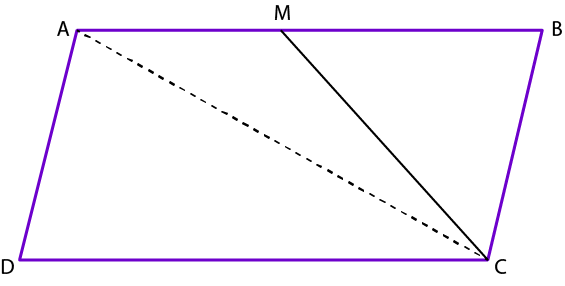From the figure we know that the diagonal AC divides the parallelogram ABCD into two triangles having the same area

It can be written as

Area of △ ADC = Area of △ ABC ……. (1)

We know that △ ADC and parallelogram ABCD are on the same base CD and between the same parallel lines DC and AM.

It can be written as

Area of △ ADC = Area of △ ABC = ½ (Area of parallelogram ABCD)

From the figure we know that M is the midpoint of AB

So we get

Area of △ AMC = Area of △ BMC = ½ (Area of △ ABC) = ½ (Area of △ ADC)

It can be written as

Area of AMCD = Area of △ ADC + Area of △ AMC

By substituting the values

24 = Area of △ ADC + ½ (Area of △ ADC)

It can be written as

24 = 3/2 (Area of △ ADC)

By cross multiplication

24 × 2 = 3 × (Area of △ ADC)

On further calculation

48 = 3 × (Area of △ ADC)

So we get

Area of △ ADC = 48/3

By division

Area of △ ADC = 16 cm2

From equation (1)

Area of △ ADC = Area of △ ABC = 16 cm2

Therefore, Area of △ ABC = 16 cm2.

10. In the adjoining figure, ABCD is a quadrilateral in which diag. BD = 14cm. If AL ⊥ BD and CM ⊥ BD such that AL = 8cm and CM = 6cm, find the area of quad. ABCD.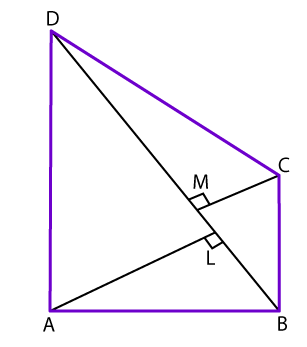Solution:

We know that

Area of △ BAD = ½ × BD × AL

By substituting the values in the above equation

Area of △ BAD = ½ × 14 × 8

By multiplication

Area of △ BAD = 56 cm2

We know that

Area of △ CBD = ½ × BD × CM

By substituting the values in the above equation

Area of △ CBD = ½ × 14 × 6

By multiplication

Area of △ CBD = 42 cm2

So we get

Area of quadrilateral ABCD = Area of △ ABD + Area of △ CBD

By substituting the values

Area of quadrilateral ABCD = 56 + 42

Area of quadrilateral ABCD = 98 cm2

Therefore, area of quadrilateral ABCD is 98 cm2.

11. If P and Q are any two points lying respectively on the sides DC and AD of a parallelogram ABCD then show that ar (△ APB) = ar (△ BQC).

Solution: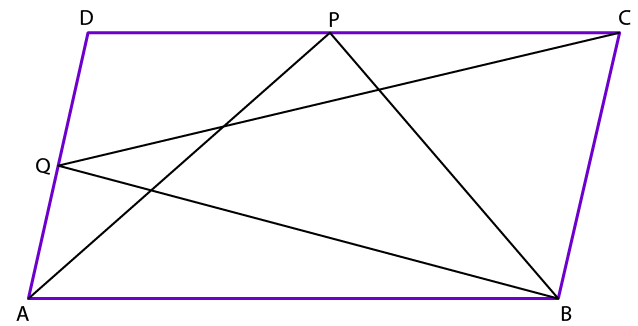From the figure we know that △ APB and parallelogram ABCD lie on the same base AB and between the same parallels AB and DC

So we get

Area of △ APB = ½ (Area of parallelogram ABCD) …….. (1)

In the same way △ BQC and parallelogram ABCD lie on the same base BC and between the same parallels BC and AD

So we get

Area of △ BQC = ½ (Area of parallelogram ABCD) …….. (1)

Using the equations (1) and (2)

We get

Area of △ APB = Area of △ BQC

Therefore, it is proved that Area of △ APB = Area of △ BQC.

12. In the adjoining figure, MNPQ and ABPQ are parallelograms and T is any point on the side BP. Prove that

(i) ar (MNPQ) = ar (ABPQ)

(ii) ar (△ ATQ) = ½ ar (MNPQ).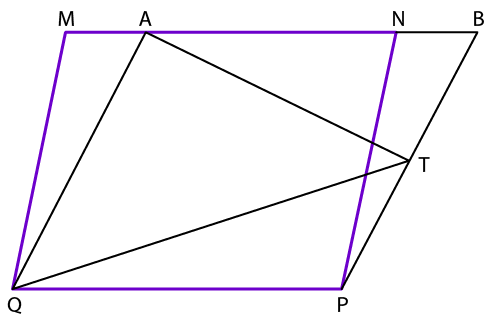Solution:

(i)From the figure we know that the parallelograms MNPQ and ABPQ are on the same base PQ and lie between the same parallels PQ and MB.

So we get

Area of parallelogram MNPQ = Area of parallelogram ABPQ

Therefore, it is proved that ar (MNPQ) = ar (ABPQ).

(ii) From the figure we know that △ ATQ and parallelogram ABPQ lie on the same base AQ and between the same parallels AQ and BP.

So we get

Area of △ ATQ = ½ (Area of parallelogram ABPQ)

It can be written as

Area of △ ATQ = ½ (Area of parallelogram MNPQ)

Therefore, it is proved that ar (△ ATQ) = ½ ar (MNPQ).

13. In the adjoining figure, ABCD is a trapezium in which AB || DC and its diagonals AC and BD intersect at O. Prove that ar (△ AOD) = ar (△ BOC).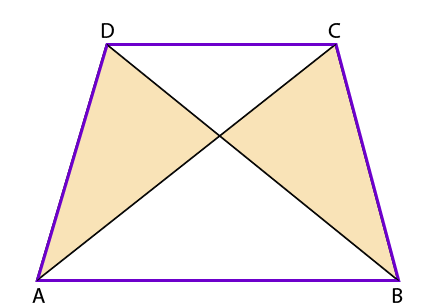Solution:

From the figure we know that △ AOD and △ DCB lie on the same base CD and between two parallel lines DC and AB.

We know that the triangles lying on the same base and parallels have equal area.

Consider △ CDA and △ CDB

It can be written as

Area of △ CDA = Area of △ CDB

So we get

Area of △ AOD = Area of △ ADC – Area of △ OCD

In the same way

Area of △ BOC = Area of △ CDB – Area of △ OCD

So we get

Area of △ BOC = Area of △ ADC – Area of △ OCD

Area of △ AOD = Area of △ BOC

Therefore, it is proved that ar (△ AOD) = ar (△ BOC).

14. In the adjoining figure, DE || BC. Prove that

(i) ar (△ ACD) = ar (△ ABE)

(ii) ar (△ OCE) = ar (△ OBD).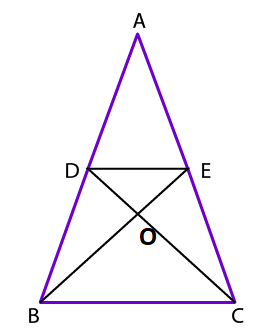Solution:

(i)From the figure we know that △ DBE and △ DCE lie on the same base DE between the parallel lines BC and DE.

So we get

Area of △ DBE = Area of △ DCE ……. (1)

Area of △ DBE + Area of △ ADE = Area of △ DCE + Area of △ ADE

We get

Area of △ ABE = Area of △ ACD

Therefore, it is proved that ar (△ ACD) = ar (△ ABE).

(ii) Subtracting △ ODE from equation (1)

We get

Area of △ DBE – Area of △ ODE = Area of △ DCE – Area of △ ODE

We get

Area of △ OBD = Area of △ OCE

Therefore, it is proved that ar (△ OCE) = ar (△ OBD).

15. Prove that a median divides a triangle into two triangles of equal area.

Solution:

Consider △ ABD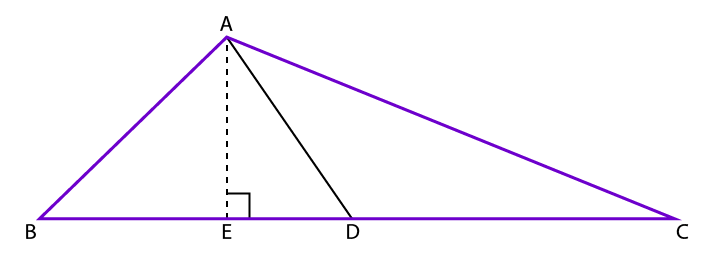We know that

Area of △ ABD = ½ × BD × AE

In the same way

Area of △ ADC = ½ × DC × AE

From the figure we know that D is the median

So we get

BD = DC

We know that

Area of △ ABD = ½ × BD × AE

It can be written as

Area of △ ABD = ½ × DC × AE

So we get

Area of △ ABD = Area of △ ADC

Therefore, it is proved that a median divides a triangle into two triangles of equal area.

16. Show that a diagonal divides a parallelogram into two triangles of equal area.

Solution: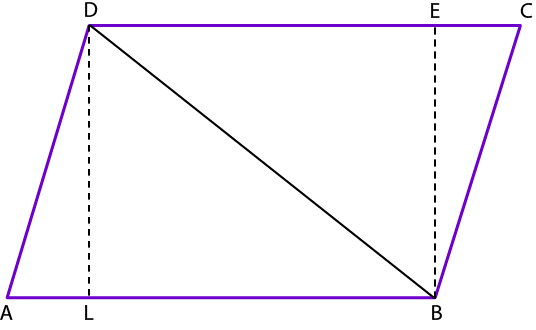We know that

Area of △ ABD = ½ × AB × DL …….. (1)

Area of △ CBD = ½ × CD × BE …….. (2)

Hence, ABCD is a parallelogram.

It can be written as AB || CD and

AB = CD …… (3)

We know that the distance between two parallel lines is constant t,

So we get

DL = BE …….. (4)

Using the equations (1), (2), (3) and (4)

We get

Area of △ ABD = ½ × AB × DL

It can be written as

Area of △ ABD = ½ × CD × BE

So we get

Area of △ ABD = Area of △ CBD

Therefore, it is proved that a diagonal divides a parallelogram into two triangles of equal area.

17. In the adjoining figure, ABC and ABD are two triangles on the same base AB. If line segment CD is bisected by AB at O, show that ar (△ ABC) = ar (△ ABD).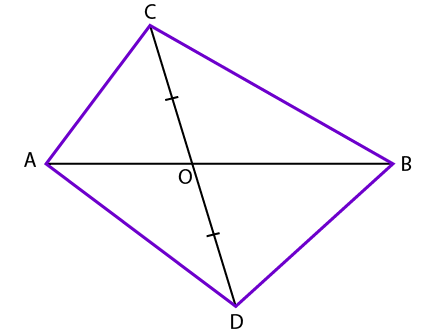Solution:

From the figure we know that median of a triangle divides it into triangles of equal area

We know that AO is the median of △ ACD

It can be written as

Area of △ COA = Area of △ DOA ……. (1)

We know that BO is the median of △ BCD

It can be written as

Area of △ COB = Area of △ DOB ……. (2)

Area of △ COA + Area of △ COB = Area of △ DOA + Area of △ DOB

So we get

Area of △ ABC = Area of △ ABD

Therefore, it is proved that ar (△ ABC) = ar (△ ABD).

18. D and E are points on sides AB and AC respectively of △ ABC such that ar (△ BCD) = ar (△ BCE). Prove that DE || BC.

Solution: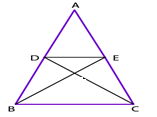From the figure we know that △ BCD and △ BCE have equal area and lie on the same base BC.

We know that

Altitude from D of △ BCD = Altitude from E of △ BCE

So we know that △ BCD and △ BCE lie between the same parallel lines

We get

DE || BC

Therefore, it is proved that DE || BC.

19. P is any point on the diagonal AC of a parallelogram ABCD. Prove that ar (△ ADP) = ar (△ ABP).

Solution:

Join the diagonal BD.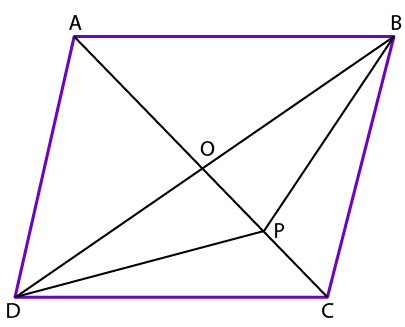From the figure we know that AC and BD are the diagonals intersecting at point O.

We know that the diagonals of a parallelogram bisect each other.

Thus, we get O as the midpoint of AC and BD.

Median of triangle divides it into two triangles having equal area.

Consider △ ABD

We know that OA is the median

So we get

Area of △ AOD = Area of △ AOB ……. (1)

Consider △ BPD

We know that OP is the median

So we get

Area of △ OPD = Area of △ OPB …….. (2)

By adding both the equations we get

Area of △ AOD + Area of △ OPD = Area of △ AOB + Area of △ OPB

So we get

Area of △ ADP = Area of △ ABP

Therefore, it is proved that ar (△ ADP) = ar (△ ABP).

20. In the adjoining figure, the diagonals AC and BD of a quadrilateral ABCD intersect at O. If BO = OD, prove that ar (△ ABC) = ar (△ ADC).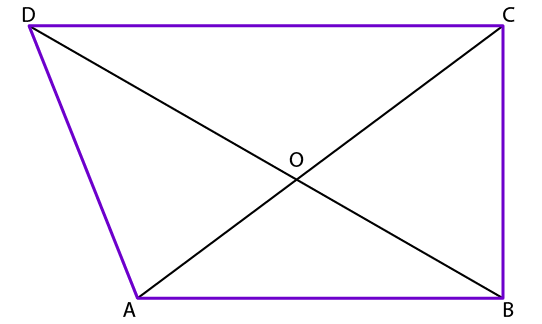Solution:

It is given that BO = OD

From the figure we know that AO is the median of △ ABD

So we get

Area of △ AOD = Area of △ AOB ……. (1)

We know that OC is the median of △ CBD

So we get

Area of △ DOC = Area of △ BOC ……. (2)

Area of △ AOD + Area of △ DOC = Area of △ AOB + Area of △ BOC

So we get

Area of △ ADC = Area of △ ABC

Therefore, it is proved that ar (△ ABC) = ar (△ ADC).

21. The vertex A of △ ABC is joined to a point D on the side BC. The midpoint of AD is E. Prove that ar (△ BEC) = ½ ar (△ ABC).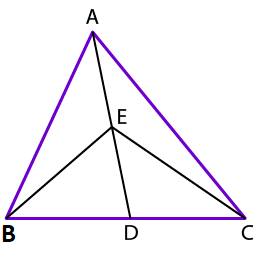Solution:

From the figure we know that BE is the median of △ ABD

So we get

Area of △ BDE = Area of △ ABE

It can be written as

Area of △ BDE = ½ (Area of △ ABD) …… (1)

From the figure we know that CE is the median of △ ADC

So we get

Area of △ CDE = Area of △ ACE

It can be written as

Area of △ CDE = ½ (Area of △ ACD) …….. (2)

Area of △ BDE + Area of △ CDE = ½ (Area of △ ABD) + ½ (Area of △ ACD)

By taking ½ as common

Area of △ BEC = ½ (Area of △ ABD + Area of △ ACD)

So we get

Area of △ BEC = ½ (Area of △ ABC)

Therefore, it is proved that ar (△ BEC) = ½ ar (△ ABC).

22. D is the midpoint of side BC of △ ABC and E is the midpoint of BD. If O is the midpoint of AE, prove that ar (△ BOE) = 1/8 ar (△ ABC).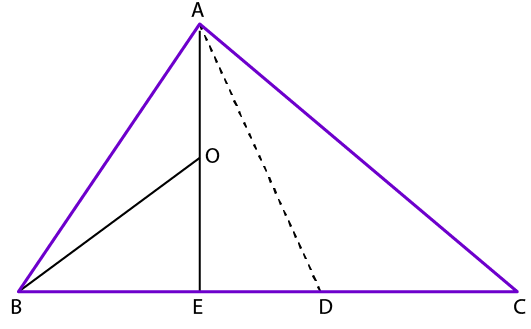Solution:

From the figure we know that O is the midpoint of AE.

We know that BO is the median of △ BAE

So we get

ar (△ BOE) = ½ ar (△ ABE) ……… (1)

We know that E is the midpoint of BD

AE divides the △ ABD into two triangles of equal area

So we get

ar (△ ABE) = ½ ar (△ ABD) ……… (2)

We know that D is the midpoint of BC

So we get

ar (△ ABD) = ½ ar (△ ABC) ……… (3)

We know that

ar (△ BOE) = ½ ar (△ ABE) using equation (1)

Substituting equation (2) in (1)

ar (△ BOE) = ½ (½ ar (△ ABD))

So we get

ar (△ BOE) = ¼ ar (△ ABD)

By substituting equation (3)

ar (△ BOE) = ¼ (½ ar (△ ABC))

So we get

ar (△ BOE) = 1/8 ar (△ ABC)

Therefore, it is proved that ar (△ BOE) = 1/8 ar (△ ABC).

23. In a trapezium ABCD, AB || DC and M is the midpoint of BC. Through M, a line PQ || AD has been drawn which meets AB in P and DC produced in Q, as shown in the adjoining figure. Prove that ar (ABCD) = ar (APQD).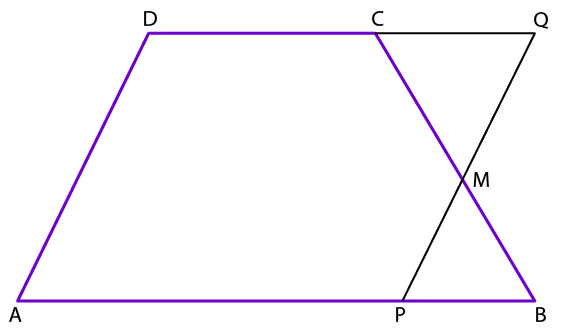Solution:

Consider △ MCQ and △ MPB

From the figure we know that

∠ QCM and ∠ PBM are alternate angles

So we get

∠ QCM = ∠ PBM

We know that M is the midpoint of BC

CM = BM

∠ CMQ and ∠ PBM are vertically opposite angles

∠ CMQ = ∠ PBM

By ASA congruence criterion

△ MCQ ≅ △ MPB

So we get

Area of △ MCQ = Area of △ MPB

We know that

Area of ABCD = Area of APQD + Area of DMPB – Area of △ MCQ

So we get

Area of ABCD = Area of APQD

Therefore, it is proved that ar (ABCD) = ar (APQD).

24. In the adjoining figure, ABCD is a quadrilateral. A line through D, parallel to AC, meets BC produced in P. Prove that ar (△ ABP) = ar (quad. ABCD).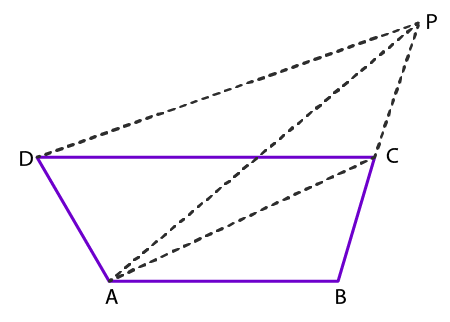Solution:

From the figure we know that △ ACP and △ ACD lie on the same base AC between parallel lines AC and DP

It can be written as

Area of △ ACP = Area of △ ACD

By adding △ ABC to both LHS and RHS

Area of △ ACP + Area of △ ABC = Area of △ ACD + Area of △ ABC

So we get

Area of △ ABP = Area of quadrilateral ABCD

Therefore, it is proved that ar (△ ABP) = ar (quad. ABCD).

25. In the adjoining figure, △ ABC and △ DBC are on the same base BC with A and D on opposite sides of BC such that ar (△ ABC) = ar (△ DBC). Show that BC bisects AD.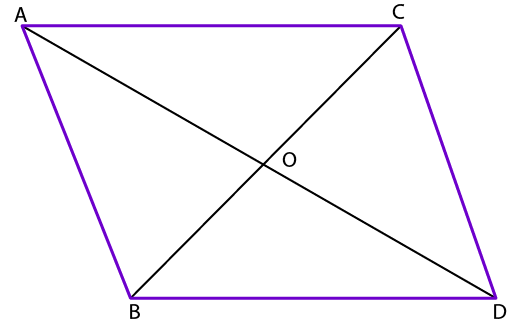Solution:

Construct AP ⊥ BC and DQ ⊥ BC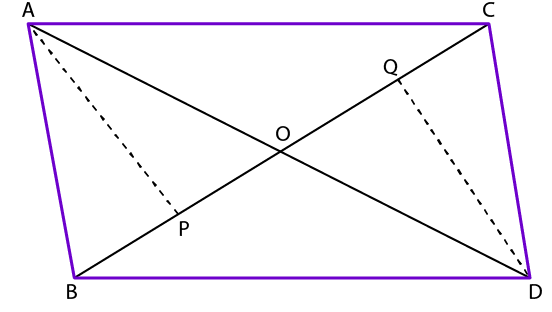We know that

Area of △ ABC = ½ × BC × AP

In the same way

Area of △ BCD = ½ × BC × DQ

Equating both we get

½ × BC × AP = ½ × BC × DQ

So we get

AP = DQ ……. (1)

Consider △ AOP and △ QOD

From the figure we know that

∠ APO = ∠ DQO = 90o

We know that ∠ AOP and ∠ DOQ are vertically opposite angles

∠ AOP = ∠ DOQ

By AAS congruence criterion

△ AOP ≅ △ QOD

OA = OD (c. p. c. t)

Therefore, it is proved that BC bisects AD.

26. ABCD is a parallelogram in which BC is produced to P such that CP = BC, as shown in the adjoining figure. AP intersects CD at M. If ar (DMB) = 7 cm2, find the area of parallelogram ABCD.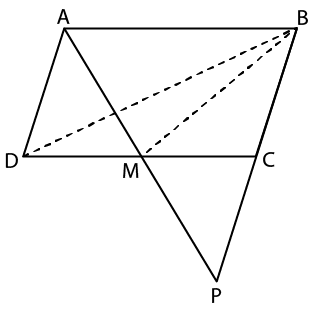Solution:

Consider △ ADM and △ PCM

From the figure we know that ∠ ADM and ∠ PCM are alternate angles

We know that AD = BC = CP

It can be written as

∠ AMD and ∠ PMC are vertically opposite angles

∠ AMD = ∠ PMC

By ASA congruence criterion

So we get

Area of △ ADM = Area of △ PCM

DM = CM (c. p. c. t)

We know that BM is the median of △ BDC

So we get

Area of △ DMB = Area of △ CMB

We get

Area of △ BDC = 2 (Area of △ DMB)

By substituting the value

Area of △ BDC = 2 × 7

By multiplication

Area of △ BDC = 14 cm2

We know that

Area of parallelogram ABCD = 2 (Area of △ BDC)

So we get

Area of parallelogram ABCD = 2 × 14

By multiplication

Area of parallelogram ABCD = 28 cm2

Therefore, area of parallelogram ABCD is 28 cm2.

27. In a parallelogram ABCD, any point E is taken on the side BC. AE and DC when produced to meet at a point M. Prove that ar (△ ADM) = ar (ABMC).

Solution:

Construct lines AC and BM.

Let us take h as the distance between AB and CD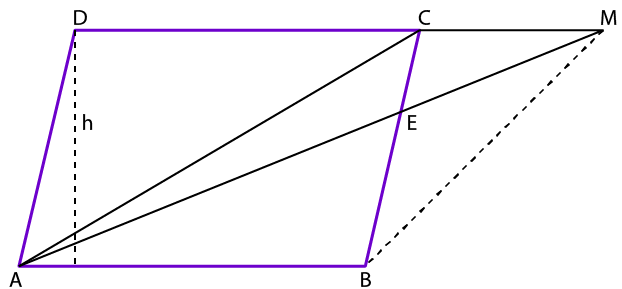We know that

Area of △ ACD = ½ × CD × h

In the same way

Area of △ ABM = ½ × AB × h

From the figure we know that AB = CD

So it can be written as

Area of △ ABM = ½ × CD × h

So we get

Area of △ ABM = Area of △ ACD

Let us add △ ACM on both sides

Area of △ ABM + Area of △ ACM = Area of △ ACD + Area of △ ACM

So we get

Area of ABMC = Area of △ ADM

Therefore, it is proved that ar (△ ADM) = ar (ABMC).

28. P, Q, R, S are respectively the midpoints of the sides AB, BC, CD and DA of parallelogram ABCD. Show that PQRS is a parallelogram and also show that ar (||gm PQRS) = ½ × ar (||gm ABCD).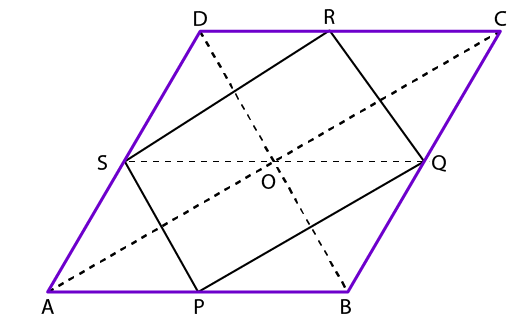Solution:

We know that

Area of parallelogram PQRS = ½ (Area of parallelogram ABCD)

Construct diagonals AC, BD and SQ

From the figure we know that S and R are the midpoints of AD and CD

Using the midpoint theorem

SR || AC

From the figure we know that P and Q are the midpoints of AB and BC

Consider △ ABC

Using the midpoint theorem

PQ || AC

It can be written as

PQ || AC || SR

So we get

PQ || SR

In the same way we get SP || RQ

Consider △ ABD

We know that O is the midpoint of AC and S is the midpoint of AD

Using the midpoint theorem

OS || AB

Consider △ ABC

Using the midpoint theorem

OQ || AB

It can be written as

SQ || AB

We know that ABQS is a parallelogram

We get

Area of △ SPQ = ½ (Area of parallelogram ABQS) ……. (1)

In the same way we get

Area of △ SRQ = ½ (Area of parallelogram SQCD) …….. (2)

Area of △ SPQ + Area of △ SRQ = ½ (Area of parallelogram ABQS + Area of parallelogram SQCD)

So we get

Area of parallelogram PQRS = ½ (Area of parallelogram ABCD)

Therefore, it is proved that ar (||gm PQRS) = ½ × ar (||gm ABCD).

29. In a triangle ABC, the medians BE and CF intersect at G. Prove that ar (△ BCG) = ar (AFGE).

Solution: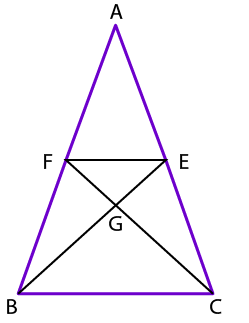Draw a line EF.

We know that the line segment joining the midpoint of two sides of a triangle is parallel to the third side.

So we get

FE || BC

From the figure we know that △ BEF and △ CEF lie on the same base EF between the same parallel lines

So we get

Area of △ BEF = Area of △ CEF

By subtracting △ GEF both the sides

Area of △ BEF – Area of △ GEF = Area of △ CEF – Area of △ GEF

We get

Area of △ BFG = Area of △ CEG …… (1)

Median of a triangle divides it into two triangles having equal area

Area of △ BEC = Area of △ ABE

It can be written as

Area of △ BGC + Area of △ CEG = Area of quadrilateral AFGE + Area of Area of △ BFG

Using the equation (1) we get

Area of △ BGC + Area of △ BFG = Area of quadrilateral AFGE + Area of Area of △ BFG

We get

Area of △ BGC = Area of quadrilateral AFGE

Therefore, it is proved that ar (△ BCG) = ar (AFGE).

30. The base BC of △ ABC is divided at D such that BD = ½ DC. Prove that ar (△ ABD) = 1/3 × ar (△ ABC).

Solution:

Construct AE ⊥ BC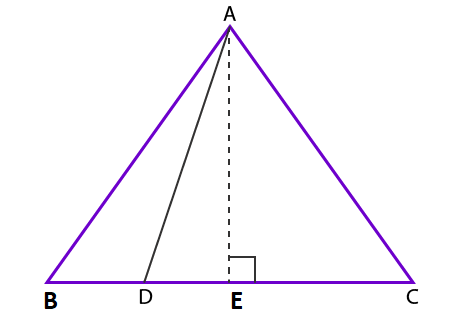We know that

Area of △ ABD = ½ × BD × AE ……. (1)

Area of △ ABC = ½ × BC × AE …….. (2)

It is given that

BD = ½ BC

We get

BC = BC + DC

It can be written as

BC = BD + 2BD

So we get

BC = 3BD

By division

BD = 1/3 BC …….. (3)

Using equation (1) we get

Area of △ ABD = ½ × BD × AE

By substituting (3)

Area of △ ABD = ½ × 1/3 × BC × AE

So we get

Area of △ ABD = 1/3 (1/2 × BC × AE)

Substituting equation (2)

Area of △ ABD = 1/3 × Area of △ ABC

Therefore, it is proved that ar (△ ABD) = 1/3 × ar (△ ABC).

31. In the adjoining figure, BD || CA, E is the midpoint of CA and BD = ½ CA. Prove that ar (△ ABC) = 2 ar (△ DBC).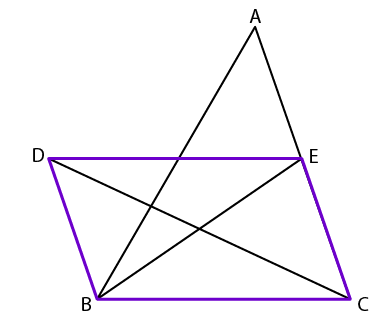Solution:

It is given that E is the midpoint of CA and BD = ½ CA

So we get

BD = CE

We know that BD || CA

BD = CE

In the same way BD || CE

BD = CE

Hence, BCED is a parallelogram.

We know that △ DBC and △ EBC lie on the same base and between the same parallel lines

So we get

Area of △ DBC = Area of △ EBC ….. (1)

From the figure we know that BE is the median of △ ABC

We get

Area of △ BEC = ½ (Area of △ ABC)

Using equation (1) we get

Area of △ DBC = ½ (Area of △ ABC)

So we get

Area of △ ABC = 2 (Area of △ DBC)

Therefore, it is proved that ar (△ ABC) = 2 ar (△ DBC).

32. The given figure shows a pentagon ABCDE. EG, drawn parallel to DA, meets BA produced to G, and CF, drawn parallel to DB, meets AB produced at F. Show that ar (pentagon ABCDE) = ar (△ DGF).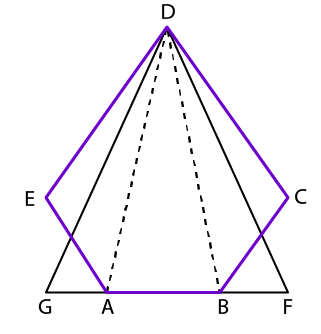Solution:

Consider △ DGA and △ AED

We know that both the triangles have the same base AD and lie between the parallel lines AD and EG.

So we get

Area of △ DGA = Area of △ AED ……. (1)

Consider △ DBC and △ BFD

We know that both the triangles have the same base DB and lie between the parallel lines BD and CF.

So we get

Area of △ DBF = Area of △ BCD ……. (2)

Area of △ DGA + Area of △ DBF = Area of △ AED + Area of △ BCD

By adding △ ABD both sides

Area of △ DGA + Area of △ DBF + Area of △ ABD = Area of △ AED + Area of △ BCD + Area of △ ABD

So we get

Area of △ DGF = Area of pentagon ABCDE

Therefore, it is proved that ar (pentagon ABCDE) = ar (△ DGF).

33. In the adjoining figure, CE || AD and CF || BA. Prove that ar (△ CBG) = ar (△ AFG).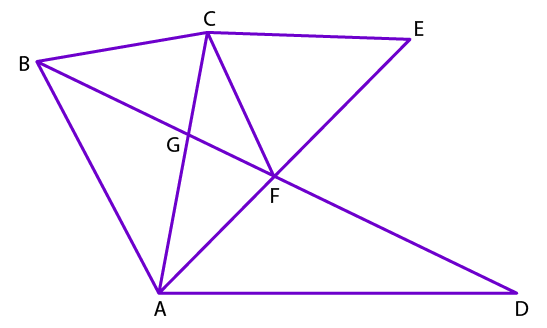Solution:

From the figure we know that △ BCF and △ ACF lie on the same base CF between the same parallels CF and BA

So we get

Area of △ BCF = Area of △ ACF

By subtracting △ CGF both sides

Area of △ BCF – Area of △ CGF = Area of △ ACF – Area of △ CGF

So we get

Area of △ CBG = Area of △ AFG

Therefore, it is proved that ar (△ CBG) = ar (△ AFG).

34. In the adjoining figure, the point D divides the side BC of △ ABC in the ratio m: n. Prove that ar (△ ABD): ar (△ ADC) = m: n.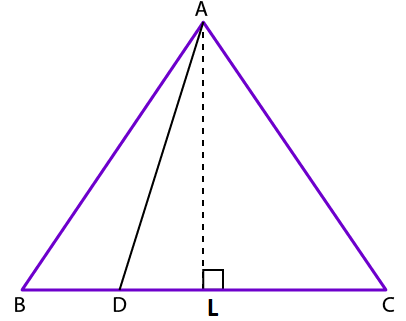Solution:

We know that

Area of △ ABD = ½ × BD × AL

Area of △ ADC = ½ × DC × AL

It is given that BD: DC = m: n

It can be written as

BD = DC × m/n

We know that

Area of △ ABD = ½ × BD × AL

By substituting BD

Area of △ ABD = ½ × (DC × m/n) × AL

So we get

Area of △ ABD = m/n × (1/2 × DC × AL)

It can be written as

Area of △ ABD = m/n × (Area of △ ADC))

We know that

Area of △ ABD/ Area of △ ADC = m/n

We can write it as

Area of △ ABD: Area of △ ADC = m: n

Therefore, it is proved that ar (△ ABD): ar (△ ADC) = m: n.

35. In a trapezium ABCD, AB || DC, AB = a cm, and DC = b cm. If M and N are the midpoints of the nonparallel sides, AD and BC respectively then find the ratio of ar (DCNM) and ar (MNBA).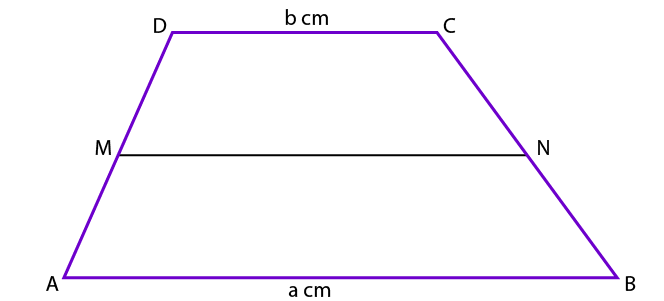Solution:

Join the diagonal DB which cuts the line MN at the point Y.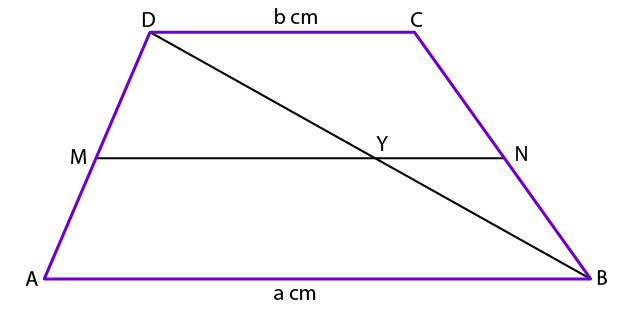We know that M and N are the midpoints of AD and BC

So we get

MN || AB || CD

We know that M is the midpoint of AD and MY || AB

So we get Y as the midpoint of DB

It can be written as

MY = ½ AB

Consider △ BDC

We know that

NY = ½ CD

We get

MN = MY + YN

By substituting the values

MN = ½ AB + ½ CD

By taking out ½ as common

MN = ½ (AB + CD)

It is given that AB = a and CD = b

So we get

MN = (a + b)/2

Construct DQ ⊥ AB where DQ cuts the line MN at the point P

So we know that P is the midpoint of DQ

It can be written as

DP = PQ = h

We get

Area of trapezium DCNM = ½ × (MN + CD) × DP

By substituting

Area of trapezium DCNM =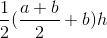So we get

Area of trapezium DCNM = h/4 (a + 3b) ……. (1)

Area of trapezium MNBA = ½ × (MN + AB) × PQ

By substituting

Area of trapezium MNBA =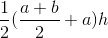So we get

Area of trapezium MNBA = h/4 (3a + b) ……. (2)

By dividing both the equations

Area of trapezium DCNM / Area of trapezium MNBA = {h/4 (a + 3b)}/ {h/4 (3a + b)}

By cancelling the similar terms

Area of trapezium DCNM / Area of trapezium MNBA = (a + 3b)/ (3a + b)

It can be written as

Area of trapezium DCNM: Area of trapezium MNBA = (a + 3b): (3a + b)

Therefore, ratio of ar (DCNM) and ar (MNBA) is (a + 3b): (3a + b)

36. ABCD is a trapezium in which AB || DC, AB = 16cm and DC = 24cm. If E and F are respectively the midpoints of AD and BC, prove that ar (ABFE) = 9/11 ar (EFCD).

Solution: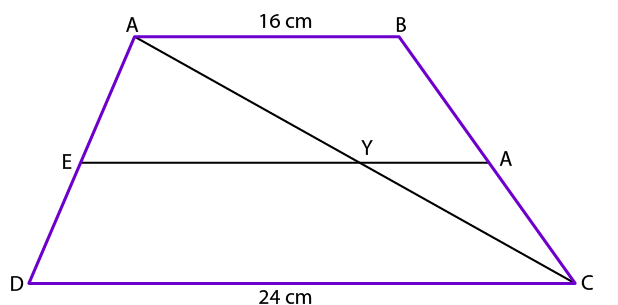Join the diagonal AC such that it cuts the line EF at the point Y

From the figure we know that E and F are the midpoints of AD and BC

So we get

EF || AB || CD

Consider △ ACF

We know that E is the midpoint of AD and EY || CD

So we get Y as the midpoint of AC

It can be written as

EY = ½ CD

Consider △ ABC

We get

FY = ½ AB

We know that

EF = EY + YF

By substituting the values

EF = ½ CD + ½ AB

By taking ½ as common

EF = ½ (CD + AB)

By substituting the values

EF = (24 + 16)/2

So we get

EF = 20cm

Construct AQ ⊥ DC such that AQ cuts EF at P

We know that P is the midpoint of AQ

So we get

AP = PQ = h

We get

Area of trapezium ABFE = 1/2 × (EF + AB) × AP

By substituting the values

Area of trapezium ABFE = ½ × (20 + 16) × h

On further calculation

Area of trapezium ABFE = 18h cm2

Area of trapezium EFCD = ½ × (EF + CD) × PQ

By substituting the values

Area of trapezium EFCD = ½ × (20 + 24) × h

On further calculation

Area of trapezium EFCD = 22h cm2

By division

Area of trapezium ABFE/ Area of trapezium EFCD = 18h/ 22h

So we get

Area of trapezium ABFE/ Area of trapezium EFCD = 9/11

It can be written as

Area of trapezium ABFE = 9/11 × (Area of trapezium EFCD)

Therefore, it is proved that ar (ABFE) = 9/11 ar (EFCD).

37. In the adjoining figure, D and E are respectively the midpoints of sides AB and AC of △ ABC. If PQ || BC and CDP and BEQ are straight lines then prove that ar (△ ABQ) = ar (△ ACP).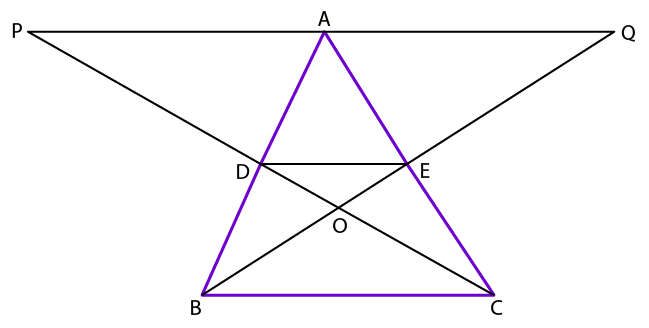Solution:

From the figure we know that D and E are the midpoints of AB and AC

So we get

DE || BC || PQ

Consider △ ACP

We know that AP || DE and E is the midpoint of AC

Using the midpoint theorem we know that D is the midpoint of PC

So we get

DE = ½ AP

It can be written as

AP = 2DE …… (1)

Consider △ ABQ

We know that AQ || DE and D is the midpoint of AB

Using the midpoint theorem we know that E is the midpoint of BQ

So we get

DE = ½ AQ

It can be written as

AQ = 2DE ……. (2)

Using equations (1) and (2)

We get

AP = AQ

We know that △ ACP and △ ABQ lie on the bases AP and AQ between the same parallels BC and PQ

So we get

Area of △ ACP = Area of △ ABQ

Therefore, it is proved that ar (△ ABQ) = ar (△ ACP).

38. In the adjoining figure, ABCD and BQSC are two parallelograms. Prove that ar (△ RSC) = ar (△ PQB).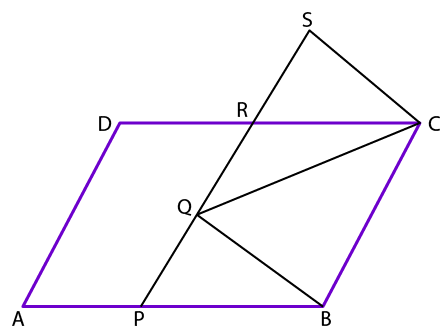Solution:

Consider △ RSC and △ PQB

From the figure we know that RC || PB and ∠ CRS and ∠ BPQ and ∠ RSC and ∠ PQB are corresponding angles

It can be written as

∠ CRS = ∠ BPQ and ∠ RSC = ∠ PQB

We know that the opposite sides of parallelogram are equal

SC = QB

By AAS congruence criterion

△ RSC ≅ △ PQB

So we get

Area of △ RSC = Area of △ PQB

Therefore, it is proved that ar (△ RSC) = ar (△ PQB).

### RS Aggarwal Solutions for Class 9 Maths Chapter 11: Areas of Parallelograms and Triangles

Chapter 11, Quadrilaterals, has 1 exercise providing solutions based on the RS Aggarwal textbook of current CBSE syllabus. Some of the topics which are explained in RS Aggarwal Solutions chapter 11 are listed below:

• Euclidean Area Axioms
• Figures on the same base and between the same parallels
• Parallelograms on the same base and between the same parallels
• Base and altitude of a parallelogram

### RS Aggarwal Solutions Class 9 Maths Chapter 11 – Areas of Parallelograms and Triangles

RS Aggarwal Solutions for class 9 is available in PDF format which can be used by the students as a source of reference. To help students have a better academic score is the main objective of preparing the solutions. The students can practise the problems on a daily basis to understand the concepts used and the methods of solving it.

The parallelograms are majorly used in industries to transfer mechanical motion from one place to another. The area of triangles are used in finding the height of objects like bridge etc. The solutions of class 9 in PDF format can be downloaded by the students and used to solve the exercise problems of chapter 11.